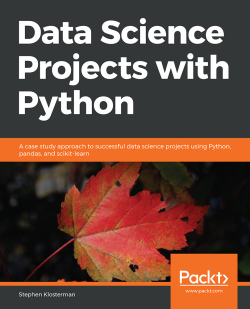Data Science Projects with Python4.6 (5 reviews total)
By Stephen Klosterman
• \$5/mo for 5 months Subscribe Access now
• \$34.99 Print + eBook
• \$24.99 eBook

• Constantly updated with 100+ new titles each month
• Breadth and depth in over 1,000+ technologies

Data Science Projects with Python is designed to give you practical guidance on industry-standard data analysis and machine learning tools, by applying them to realistic data problems. You will learn how to use pandas and Matplotlib to critically examine datasets with summary statistics and graphs, and extract the insights you seek to derive. You will build your knowledge as you prepare data using the scikit-learn package and feed it to machine learning algorithms such as regularized logistic regression and random forest. You’ll discover how to tune algorithms to provide the most accurate predictions on new and unseen data. As you progress, you’ll gain insights into the working and output of these algorithms, building your understanding of both the predictive capabilities of the models and why they make these predictions.

By then end of this book, you will have the necessary skills to confidently use machine learning algorithms to perform detailed data analysis and extract meaningful insights from unstructured data.

Publication date:
April 2019
Publisher
Packt
Pages
374
ISBN
9781838551025

Chapter 1. Data Exploration and Cleaning

Note

Learning Objectives

By the end of this chapter, you will be able to:

• Perform basic operations in Python

• Describe the business context of the case study data and its suitability for the task

• Perform data cleaning operations

• Examine statistical summaries and visualize the case study data

• Implement one-hot encoding on categorical variables

Note

This chapter will get you started with basic operations in Python and shows you how to perform data-related operations such as data verification, data cleaning, datatype conversion, examining statistical summaries, and more.

Introduction

Most businesses possess a wealth of data on their operations and customers. Reporting on these data in the form of descriptive charts, graphs, and tables is a good way to understand the current state of the business. However, in order to provide quantitative guidance on future business strategies and operations, it is necessary to go a step further. This is where the practices of machine learning and predictive modeling become involved. In this book, we will show how to go from descriptive analyses to concrete guidance for future operations using predictive models.

To accomplish this goal, we'll introduce some of the most widely-used machine learning tools via Python and many of its packages. You will also get a sense of the practical skills necessary to execute successful projects: inquisitiveness when examining data and communication with the client. Time spent looking in detail at a dataset and critically examining whether it accurately meets its intended purpose is time well spent. You will learn several techniques for assessing data quality here.

In this chapter, after getting familiar with the basic tools for data exploration, we will discuss a few typical working scenarios for how you may receive data. Then, we will begin a thorough exploration of the case study dataset and help you learn how you can uncover possible issues, so that when you are ready for modeling, you may proceed with confidence.

Python and the Anaconda Package Management System

In this book, we will use the Python programming language. Python is a top language for data science and is one of the fastest growing programming languages. A commonly cited reason for Python's popularity is that it is easy to learn. If you have Python experience, that's great; however, if you have experience with other languages, such as C, Matlab, or R, you shouldn't have much trouble using Python. You should be familiar with the general constructs of computer programming to get the most out of this book. Examples of such constructs are for loops and if statements that guide the control flow of a program. No matter what language you have used, you are likely familiar with these constructs, which you will also find in Python.

A key feature of Python, that is different from some other languages, is that it is zero-indexed; in other words, the first element of an ordered collection has an index of 0. Python also supports negative indexing, where index-1 refers to the last element of an ordered collection and negative indices count backwards from the end. The slice operator, :, can be used to select multiple elements of an ordered collection from within a range, starting from the beginning, or going to the end of the collection.

Indexing and the Slice Operator

Here, we demonstrate how indexing and the slice operator work. To have something to index, we will create a list, which is a mutable ordered collection that can contain any type of data, including numerical and string types. "Mutable" just means the elements of the list can be changed after they are first assigned. To create the numbers for our list, which will be consecutive integers, we'll use the built-in range() Python function. The range() function technically creates an iterator that we'll convert to a list using the list() function, although you need not be concerned with that detail here. The following screenshot shows a basic list being printed on the console: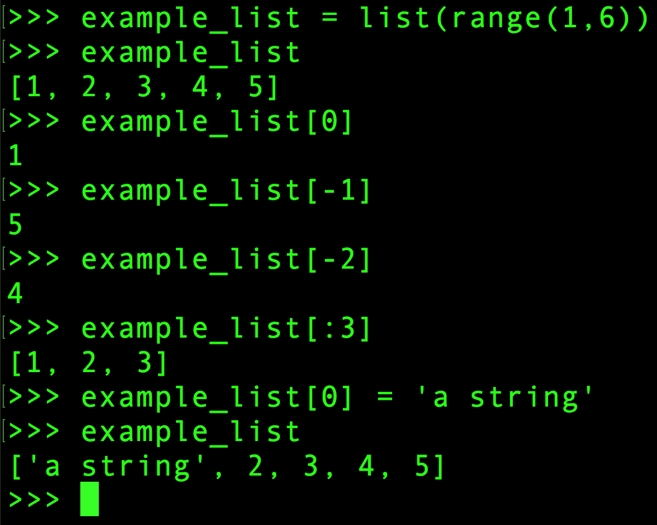Figure 1.1: List creation and indexing

A few things to notice about Figure 1.1: the endpoint of an interval is open for both slice indexing and the range() function, while the starting point is closed. In other words, notice how when we specify the start and end of range(), endpoint 6 is not included in the result but starting point 1 is. Similarly, when indexing the list with the slice [:3], this includes all elements of the list with indices up to, but not including, 3.

We've referred to ordered collections, but Python also includes unordered collections. An important one of these is called a dictionary. A dictionary is an unordered collection of key:value pairs. Instead of looking up the values of a dictionary by integer indices, you look them up by keys, which could be numbers or strings. A dictionary can be created using curly braces {} and with the key:value pairs separated by commas. The following screenshot is an example of how we can create a dictionary with counts of fruit – examine the number of apples, then add a new type of fruit and its count: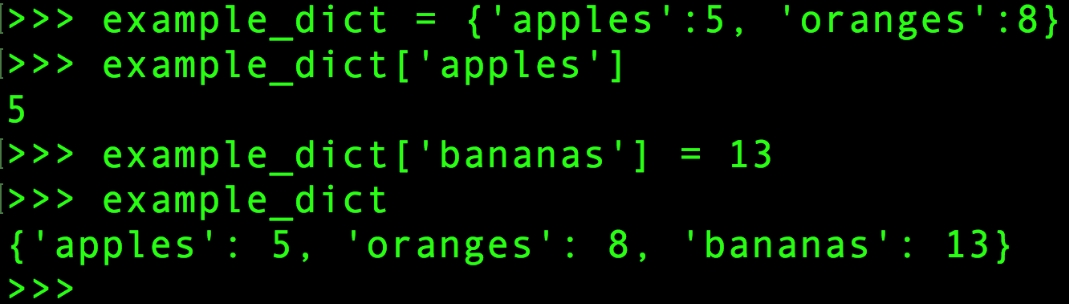Figure 1.2: An example dictionary

There are many other distinctive features of Python and we just want to give you a flavor here, without getting into too much detail. In fact, you will probably use packages such as pandas (pandas) and NumPy (numpy) for most of your data handling in Python. NumPy provides fast numerical computation on arrays and matrices, while pandas provides a wealth of data wrangling and exploration capabilities on tables of data called DataFrames. However, it's good to be familiar with some of the basics of Python—the language that sits at the foundation of all of this. For example, indexing works the same in NumPy and pandas as it does in Python.

One of the strengths of Python is that it is open source and has an active community of developers creating amazing tools. We will use several of these tools in this book. A potential pitfall of having open source packages from different contributors is the dependencies between various packages. For example, if you want to install pandas, it may rely on a certain version of NumPy, which you may or may not have installed. Package management systems make life easier in this respect. When you install a new package through the package management system, it will ensure that all the dependencies are met. If they aren't, you will be prompted to upgrade or install new packages as necessary.

For this book, we will use the Anaconda package management system, which you should already have installed. While we will only use Python here, it is also possible to run R with Anaconda.

Note

Environments

If you previously had Anaconda installed and were using it prior to this book, you may wish to create a new Python 3.x environment for the book. Environments are like separate installations of Python, where the set of packages you have installed can be different, as well as the version of Python. Environments are useful for developing projects that need to be deployed in different versions of Python. For more information, see https://conda.io/docs/user-guide/tasks/manage-environments.html.

Exercise 1: Examining Anaconda and Getting Familiar with Python

In this exercise, you will examine the packages in your Anaconda installation and practice some basic Python control flow and data structures, including a for loop, dict, and list. This will confirm that you have completed the installation steps in the preface and show you how Python syntax and data structures may be a little different from other programming languages you may be familiar with. Perform the following steps to complete the exercise:

Note

The code file for this exercise can be found here: http://bit.ly/2Oyag1h.

1. Open up a Terminal, if you're using macOS or Linux, or a Command Prompt window in Windows. Type conda list at the command line. You should observe an output similar to the following: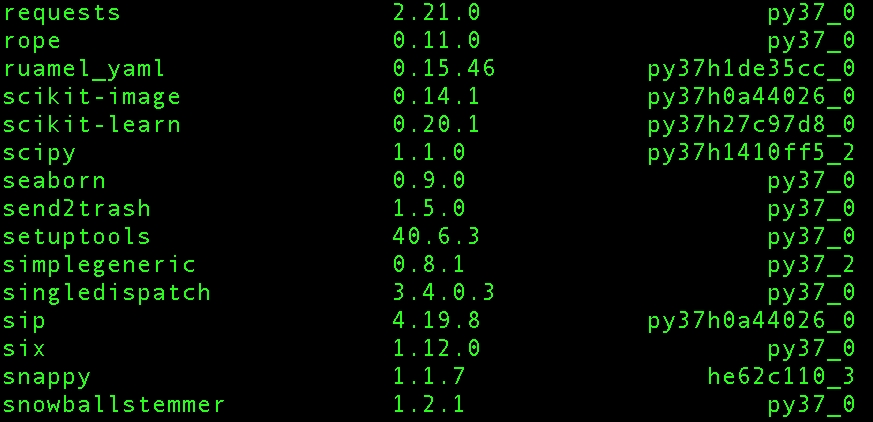Figure 1.3: Selection of packages from conda list

You can see all the packages installed in your environment. Look how many packages already come with the default Anaconda installation! These include all the packages we will need for the book. However, installing new packages and upgrading existing ones is easy and straightforward with Anaconda; this is one of the main advantages of a package management system.

2. Type python in the Terminal to open a command-line Python interpreter. You should obtain an output similar to the following: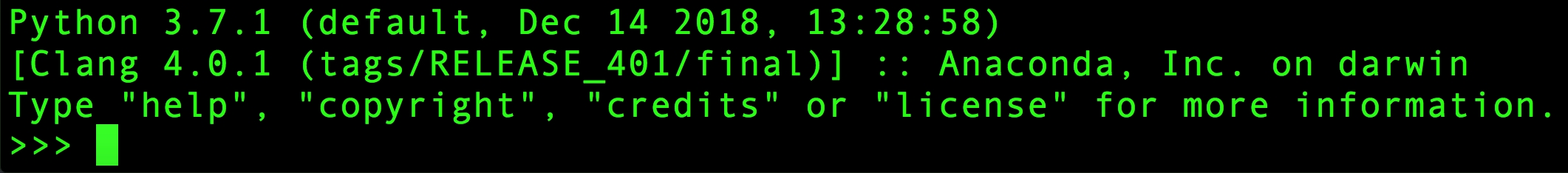Figure 1.4: Command-line Python

You should also see some information about your version of Python, as well as the Python command prompt (>>>). When you type after this prompt, you are writing Python code.

Note

Although we will be using the Jupyter Notebook in this book, one of the aims of this exercise is to go through the basic steps of writing and running Python programs on the command prompt.

3. Write a for loop at the command prompt to print values from 0 to 4 using the following code:

for counter in range(5):
...    print(counter)
...

Once you hit Enter when you see (...) on the prompt, you should obtain this output: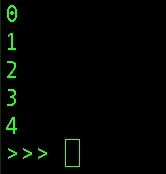Figure 1.5: Output of a for loop at the command line

Notice that in Python, the opening of the for loop is followed by a colon, and the body of the loop requires indentation. It's typical to use four spaces to indent a code block. Here, the for loop prints the values returned by the range() iterator, having repeatedly accessed them using the counter variable with the in keyword.

Note

For many more details on Python code conventions, refer to the following: https://www.python.org/dev/peps/pep-0008/.

Now, we will return to our dictionary example. The first step here is to create the dictionary.

4. Create a dictionary of fruits (apples, oranges, and bananas) using the following code:

example_dict = {'apples':5, 'oranges':8, 'bananas':13}
5. Convert the dictionary to a list using the list() function, as shown in the following snippet:

dict_to_list = list(example_dict)
dict_to_list

Once you run the preceding code, you should obtain the following output: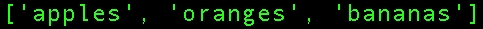Figure 1.6: Dictionary keys converted to a list

Notice that when this is done and we examine the contents, only the keys of the dictionary have been captured in the list. If we wanted the values, we would have had to specify that with the .values() method of the list. Also, notice that the list of dictionary keys happens to be in the same order that we wrote them in when creating the dictionary. This is not guaranteed, however, as dictionaries are unordered collection types.

One convenient thing you can do with lists is to append other lists to them with the + operator. As an example, in the next step we will combine the existing list of fruit with a list that contains just one more type of fruit, overwriting the variable containing the original list.

6. Use the + operator to combine the existing list of fruits with a new list containing only one fruit (pears):

dict_to_list = dict_to_list + ['pears']
dict_to_list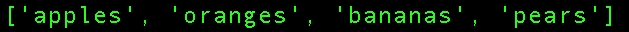Figure 1.7: Appending to a list

What if we wanted to sort our list of fruit types?

Python provides a built-in sorted() function that can be used for this; it will return a sorted version of the input. In our case, this means the list of fruit types will be sorted alphabetically.

7. Sort the list of fruits in alphabetical order using the sorted() function, as shown in the following snippet:

sorted(dict_to_list)

Once you run the preceding code, you should see the following output: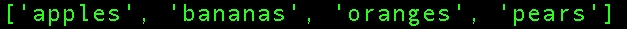Figure 1.8: Sorting a list

That's enough Python for now. We will show you how to execute the code for this book, so your Python knowledge should improve along the way.

Note

As you learn more and inevitably want to try new things, you will want to consult the documentation: https://docs.python.org/3/.

Different Types of Data Science Problems

Much of your time as a data scientist is likely to be spent wrangling data: figuring out how to get it, getting it, examining it, making sure it's correct and complete, and joining it with other types of data. pandas will facilitate this process for you. However, if you aspire to be a machine learning data scientist, you will need to master the art and science of predictive modeling. This means using a mathematical model, or idealized mathematical formulation, to learn the relationships within the data, in the hope of making accurate and useful predictions when new data comes in.

For this purpose, data is typically organized in a tabular structure, with features and a response variable. For example, if you want to predict the price of a house based on some characteristics about it, such as area and number of bedrooms, these attributes would be considered the features and the price of the house would be the response variable. The response variable is sometimes called the target variable or dependent variable, while the features may also be called the independent variables.

If you have a dataset of 1,000 houses including the values of these features and the prices of the houses, you can say you have 1,000 samples of labeled data, where the labels are the known values of the response variable: the prices of different houses. Most commonly, the tabular data structure is organized so that different rows are different samples, while features and the response occupy different columns, along with other metadata such as sample IDs, as shown in Figure 1.9.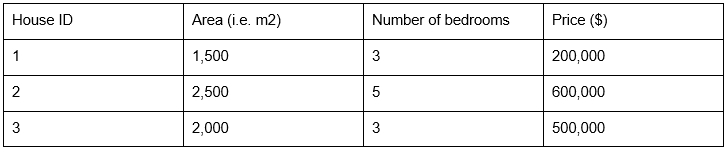Figure 1.9: Labeled data (the house prices are the known target variable)

Regression Problem

Once you have trained a model to learn the relationship between the features and response using your labeled data, you can then use it to make predictions for houses where you don't know the price, based on the information contained in the features. The goal of predictive modeling in this case is to be able to make a prediction that is close to the true value of the house. Since we are predicting a numerical value on a continuous scale, this is called a regression problem.

Classification Problem

On the other hand, if we were trying to make a qualitative prediction about the house, to answer a yes or no question such as "will this house go on sale within the next five years?" or "will the owner default on the mortgage?", we would be solving what is known as a classification problem. Here, we would hope to answer the yes or no question correctly. The following figure is a schematic illustrating how model training works, and what the outcomes of regression or classification models might be: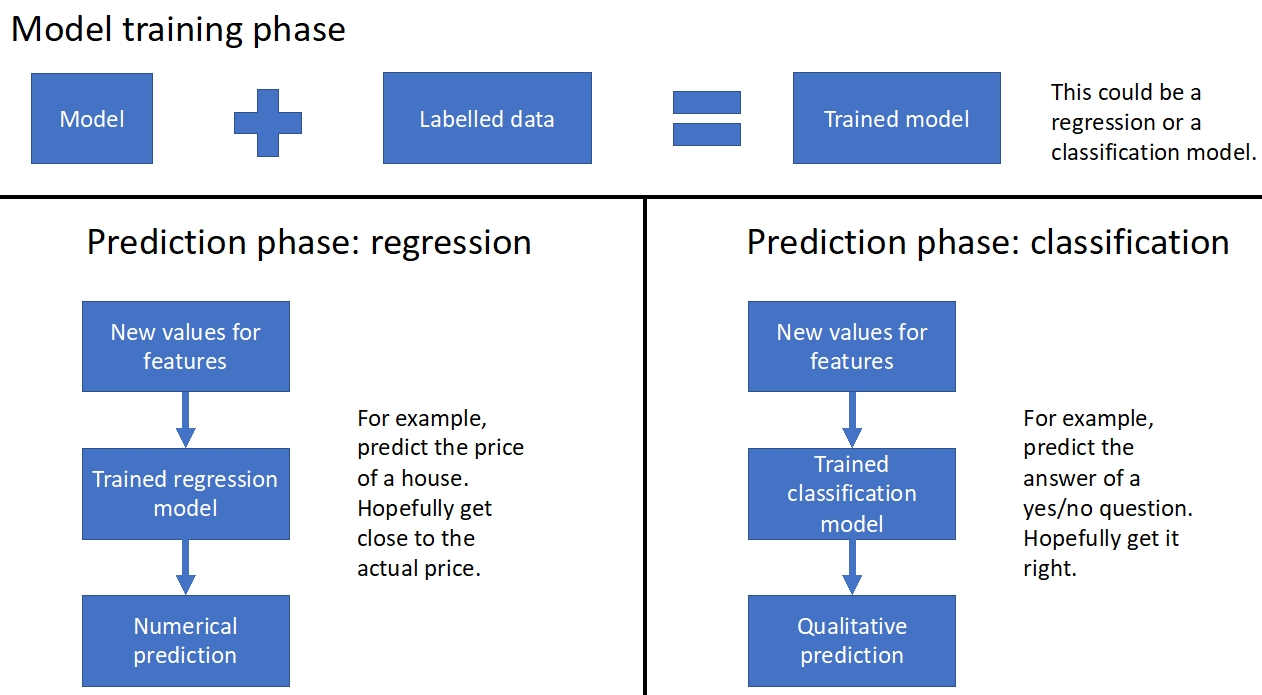Figure 1.10: Schematic of model training and prediction for regression and classification

Classification and regression tasks are called supervised learning, which is a class of problems that relies on labeled data. These problems can be thought of a needing "supervision" by the known values of the target variable. By contrast, there is also unsupervised learning, which relates to more open-ended questions of trying to find some sort of structure in a dataset that does not necessarily have labels. Taking a broader view, any kind of applied math problem, including fields as varied as optimization, statistical inference, and time series modeling, may potentially be considered an appropriate responsibility for a data scientist.

Now it's time to take a first look at the data we will use in our case study. We won't do anything in this section other than ensure that we can load the data into a Jupyter Notebook correctly. Examining the data, and understanding the problem you will solve with it, will come later.

The data file is an Excel spreadsheet called default_of_credit_card_clients__courseware_version_1_13_19.xls. We recommend you first open the spreadsheet in Excel, or the spreadsheet program of your choice. Note the number of rows and columns, and look at some example values. This will help you know whether or not you have loaded it correctly in the Jupyter Notebook.

Note

The dataset can obtained from the following link: http://bit.ly/2HIk5t3. This is a modified version of the original dataset, which has been sourced from the UCI Machine Learning Repository [http://archive.ics.uci.edu/ml]. Irvine, CA: University of California, School of Information and Computer Science.

What is a Jupyter Notebook?

Jupyter Notebooks are interactive coding environments that allow for in-line text and graphics. They are great tools for data scientists to communicate and preserve their results, since both the methods (code) and the message (text and graphics) are integrated. You can think of the environment as a kind of webpage where you can write and execute code. Jupyter Notebooks can, in fact, be rendered as web pages and are done so on GitHub. Here is one of our example notebooks: http://bit.ly/2OvndJg. Look it over and get a sense of what you can do. An excerpt from this notebook is displayed here, showing code, graphics, and prose, known as markdown in this context: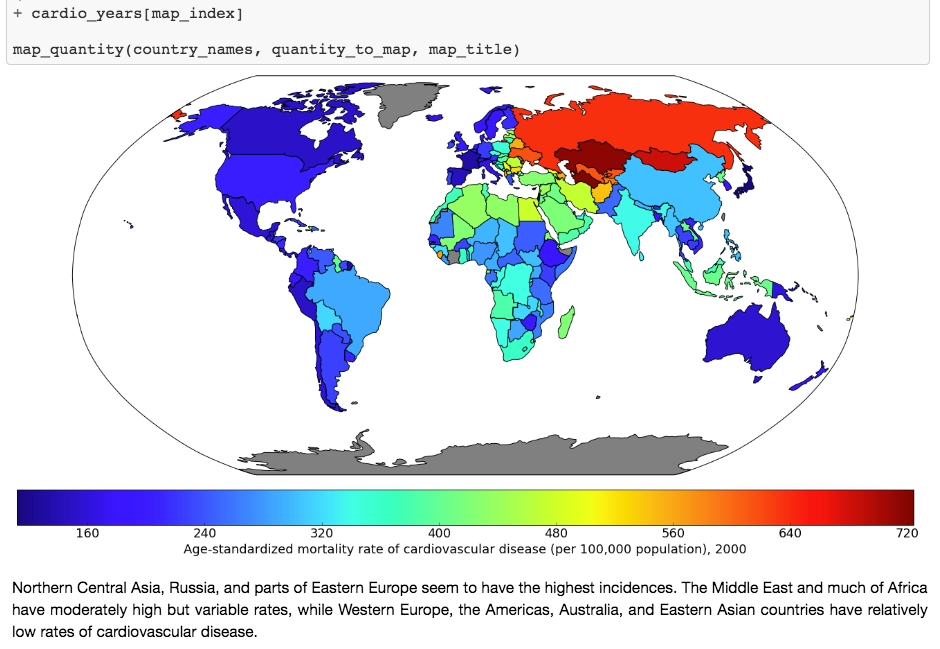Figure 1.11: Example of a Jupyter Notebook showing code, graphics, and markdown text

One of the first things to learn about Jupyter Notebooks is how to navigate around and make edits. There are two modes available to you. If you select a cell and press Enter, you are in edit mode and you can edit the text in that cell. If you press Esc, you are in command mode and you can navigate around the notebook.

When you are in command mode, there are many useful hotkeys you can use. The Up and Down arrows will help you select different cells and scroll through the notebook. If you press y on a selected cell in command mode, it changes it to a code cell, in which the text is interpreted as code. Pressing m changes it to a markdown cell. Shift + Enter evaluates the cell, rendering the markdown or executing the code, as the case may be.

Our first task in our first Jupyter Notebook will be to load the case study data. To do this, we will use a tool called pandas. It is probably not a stretch to say that pandas is the pre-eminent data-wrangling tool in Python.

A DataFrame is a foundational class in pandas. We'll talk more about what a class is later, but you can think of it as a template for a data structure, where a data structure is something like the lists or dictionaries we discussed earlier. However, a DataFrame is much richer in functionality than either of these. A DataFrame is similar to spreadsheets in many ways. There are rows, which are labeled by a row index, and columns, which are usually given column header-like labels that can be thought of as a column index. Index is, in fact, a data type in pandas used to store indices for a DataFrame, and columns have their own data type called Series.

You can do a lot of the same things with a DataFrame that you can do with Excel sheets, such as creating pivot tables and filtering rows. pandas also includes SQL-like functionality. You can join different DataFrames together, for example. Another advantage of DataFrames is that once your data is contained in one of them, you have the capabilities of a wealth of pandas functionality at your fingertips. The following figure is an example of a pandas DataFrame: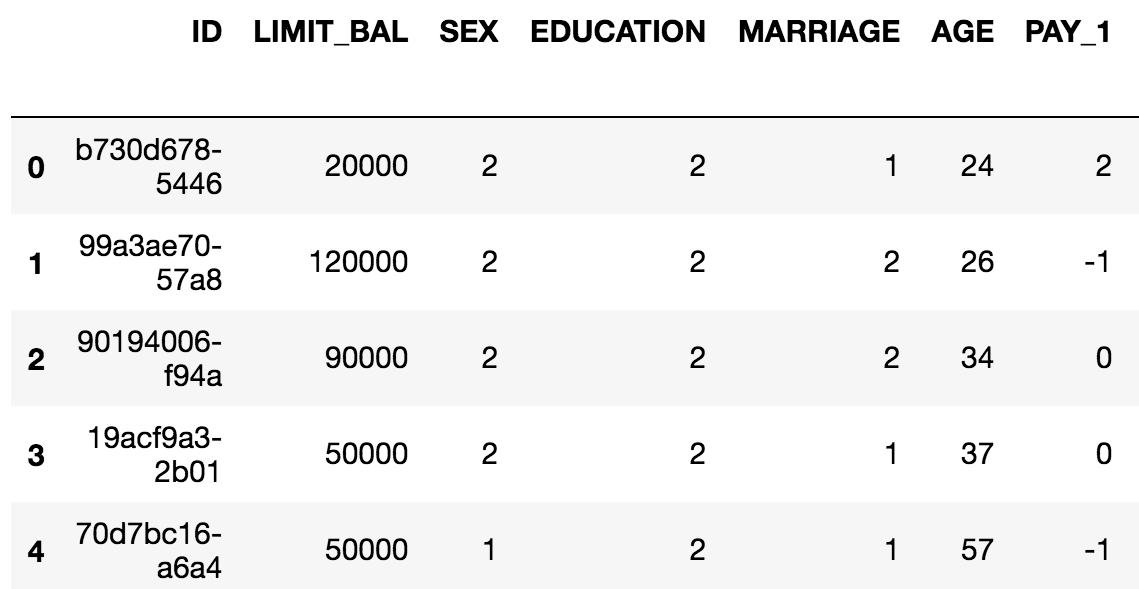Figure 1.12: Example of a pandas DataFrame with an integer row index at the left and a column index of strings

The example in Figure 1.12 is in fact the data for the case study. As a first step with Jupyter and pandas, we will now see how to create a Jupyter Notebook and load data with pandas. There are several convenient functions you can use in pandas to explore your data, including .head() to see the first few rows of the DataFrame, .info() to see all columns with datatypes, .columns to return a list of column names as strings, and others we will learn about in the following exercises.

Now that you've learned about Jupyter Notebooks, the environment in which we'll write code, and pandas, the data wrangling package, let's create our first Jupyter Notebook. We'll use pandas within this notebook to load the case study data and briefly examine it. Perform the following steps to complete the exercise:

Note

For Exercises 2–5 and Activity 1, the code and the resulting output have been loaded in a Jupyter Notebook that can be found at http://bit.ly/2W9cwPH. You can scroll to the appropriate section within the Jupyter Notebook to locate the exercise or activity of choice.

1. Open a Terminal (macOS or Linux) or a Command Prompt window (Windows) and type jupyter notebook.

You will be presented with the Jupyter interface in your web browser. If the browser does not open automatically, copy and paste the URL from the terminal in to your browser. In this interface, you can navigate around your directories starting from the directory you were in when you launched the notebook server.

2. Navigate to a convenient location where you will store the materials for this book, and create a new Python 3 notebook from the New menu, as shown here: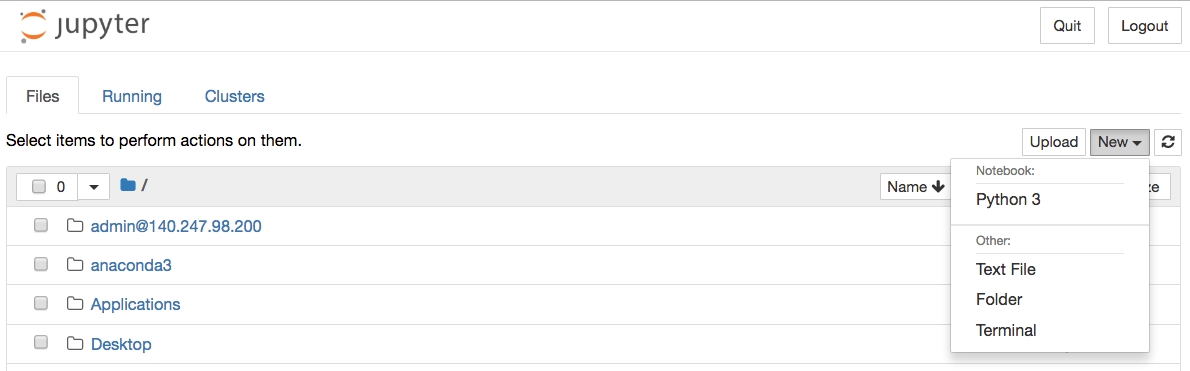Figure 1.13: Jupyter home screen

3. Make your very first cell a markdown cell by typing m while in command mode (press Esc to enter command mode), then type a number sign, #, at the beginning of the first line, followed by a space, for a heading. Make a title for your notebook here. On the next few lines, place a description.

Here is a screenshot of an example, including other kinds of markdown such as bold, italics, and the way to write code-style text in a markdown cell: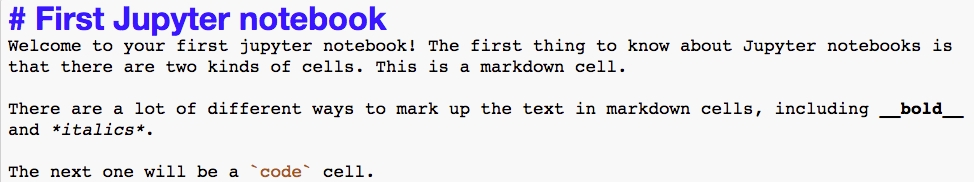Figure 1.14: Unrendered markdown cell

Note that it is good practice to make a title and brief description of your notebook, to identify its purpose to readers.

4. Press Shift + Enter to render the markdown cell.

This should also create a new cell, which will be a code cell. You can change it to a markdown cell, as you now know how to do, by pressing m, and back to a code cell by pressing y. You will know it's a code cell because of the In [ ]: next to it.

5. Type import pandas as pd in the new cell, as shown in the following screenshot: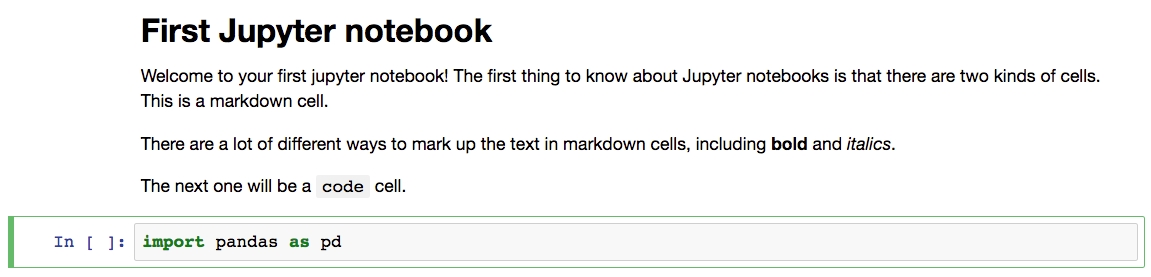Figure 1.15: Rendered markdown cell and code cell

After you execute this cell, the pandas library will be loaded into your computing environment. It's common to import libraries with "as" to create a short alias for the library. Now, we are going to use pandas to load the data file. It's in Microsoft Excel format, so we can use pd.read_excel.

Note

6. Import the dataset, which is in the Excel format, as a DataFrame using the pd.read_excel() method, as shown in the following snippet:

Note that you need to point the Excel reader to wherever the file is located. If it's in the same directory as your notebook, you could just enter the filename. The pd.read_excel method will load the Excel file into a DataFrame, which we've called df. The power of pandas is now available to us.

Let's do some quick checks in the next few steps. First, do the numbers of rows and columns match what we know from looking at the file in Excel?

7. Use the .shape method to review the number of rows and columns, as shown in the following snippet:

df.shape

Once you run the cell, you will obtain the following output: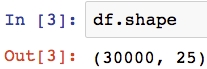Figure 1.16: Checking the shape of a DataFrame

This should match your observations from the spreadsheet. If it doesn't, you would then need to look into the various options of pd.read_excel to see if you needed to adjust something.

With this exercise, we have successfully loaded our dataset into the Jupyter Notebook. You can also have a look at the .info() and .head() methods, which will tell you information about all the columns, and show you the first few rows of the DataFrame, respectively. Now you're up and running with your data in pandas.

As a final note, while this may already be clear, observe that if you define a variable in one code cell, it is available to you in other code cells within the notebook. The code cells within a notebook share scope as long as the kernel is running, as shown in the following screenshot: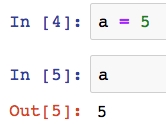Figure 1.17: Variable in scope between cells

Getting Familiar with Data and Performing Data Cleaning

Now let's imagine we are taking our first look at this data. In your work as a data scientist, there are several possible scenarios in which you may receive such a dataset. These include the following:

1. You created the SQL query that generated the data.

2. A colleague wrote a SQL query for you, with your input.

3. A colleague who knows about the data gave it to you, but without your input.

4. You are given a dataset about which little is known.

In cases 1 and 2, your input was involved in generating/extracting the data. In these scenarios, you probably understood the business problem and then either found the data you needed with the help of a data engineer, or did your own research and designed the SQL query that generated the data. Often, especially as you gain more experience in your data science role, the first step will be to meet with the business partner to understand, and refine the mathematical definition of, the business problem. Then, you would play a key role in defining what is in the dataset.

Even if you have a relatively high level of familiarity with the data, doing data exploration and looking at summary statistics of different variables is still an important first step. This step will help you select good features, or give you ideas about how you can engineer new features. However, in the third and fourth cases, where your input was not involved or you have little knowledge about the data, data exploration is even more important.

Another important initial step in the data science process is examining the data dictionary. The data dictionary, as the term implies, is a document that explains what the data owner thinks should be in the data, such as definitions of the column labels. It is the data scientist's job to go through the data carefully to make sure that these impressions match the reality of what is in the data. In cases 1 and 2, you will probably need to create the data dictionary yourself, which should be considered essential project documentation. In cases 3 and 4, you should seek out the dictionary if at all possible.

The case study data we'll use in this book is basically similar to case 3 here.

Our client is a credit card company. They have brought us a dataset that includes some demographics and recent financial data (the past six months) for a sample of 30,000 of their account holders. This data is at the credit account level; in other words, there is one row for each account (you should always clarify what the definition of a row is, in a dataset). Rows are labeled by whether in the next month after the six month historical data period, an account owner has defaulted, or in other words, failed to make the minimum payment.

Goal

Your goal is to develop a predictive model for whether an account will default next month, given demographics and historical data. Later in the book, we'll discuss the practical application of the model.

The data is already prepared, and a data dictionary is available. The dataset supplied with the book, default_of_credit_card_clients__courseware_version_1_21_19.xls, is a modified version of this dataset in the UCI Machine Learning Repository: https://archive.ics.uci.edu/ml/datasets/default+of+credit+card+clients. Have a look at that web page, which includes the data dictionary.

Note

The original dataset has been obtained from UCI Machine Learning Repository [http://archive.ics.uci.edu/ml]. Irvine, CA: University of California, School of Information and Computer Science. In this book, we have modified the dataset to suit the book objectives. The modified dataset can be found here: http://bit.ly/2HIk5t3.

Data Exploration Steps

Now that we've understood the business problem and have an idea of what is supposed to be in the data, we can compare these impressions to what we actually see in the data. Your job in data exploration is to not only look through the data both directly and using numerical and graphical summaries, but also to think critically about whether the data make sense and match what you have been told about them. These are helpful steps in data exploration:

1. How many columns are there in the data?

These may be features, response, or metadata.

2. How many rows (samples)?

3. What kind of features are there? Which are categorical and which are numerical?

Categorical features have values in discrete classes such as "Yes," "No," or "maybe."Numerical features are typically on a continuous numerical scale, such as dollar amounts.

4. What does the data look like in these features?

To see this, you can examine the range of values in numeric features, or the frequency of different classes in categorical features, for example.

5. Is there any missing data?

We have already answered questions 1 and 2 in the previous section; there are 30,000 rows and 25 columns. As we start to explore the rest of these questions in the following exercise, pandas will be our go-to tool. We begin by verifying basic data integrity in the next exercise.

Note

Note that compared to the website's description of the data dictionary, X6-X11 are called PAY_1-PAY_6 in our data. Similarly, X12-X17 are BILL_AMT1-BILL_AMT6, and X18-X23 are PAY_AMT1-PAY_AMT6.

Exercise 3: Verifying Basic Data Integrity

In this exercise, we will perform a basic check on whether our dataset contains what we expect and verify whether there are the correct number of samples.

The data are supposed to have observations for 30,000 credit accounts. While there are 30,000 rows, we should also check whether there are 30,000 unique account IDs. It's possible that, if the SQL query used to generate the data was run on an unfamiliar schema, values that are supposed to be unique are in fact not unique.

To examine this, we can check if the number of unique account IDs is the same as the number of rows. Perform the following steps to complete the exercise:

Note

The code and the resulting output graphics for this exercise have been loaded in a Jupyter Notebook that can be found here: http://bit.ly/2W9cwPH.

1. Examine the column names by running the following command in the cell:

df.columns

The .columns method of the DataFrame is employed to examine all the column names. You will obtain the following output once you run the cell: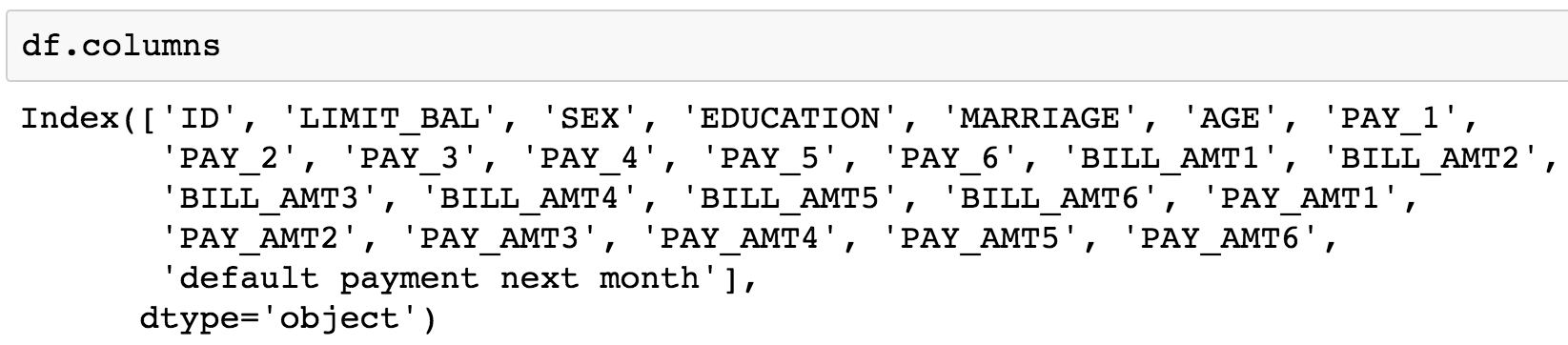Figure 1.18: Columns of the dataset

As can be observed, all column names are listed in the output. The account ID column is referenced as ID. The remaining columns appear to be our features, with the last column being the response variable. Let's quickly review the dataset information that was given to us by the client:

LIMIT_BAL: Amount of the credit provided (in New Taiwanese (NT) dollar) including individual consumer credit and the family (supplementary) credit.

SEX: Gender (1 = male; 2 = female).

Note

We will not be using the gender data to decide credit-worthiness owing to ethical considerations.

EDUCATION: Education (1 = graduate school; 2 = university; 3 = high school; 4 = others).

MARRIAGE: Marital status (1 = married; 2 = single; 3 = others).

AGE: Age (year).

PAY_1Pay_6: A record of past payments. Past monthly payments, recorded from April to September, are stored in these columns.

PAY_1 represents the repayment status in September; PAY_2 = repayment status in August; and so on up to PAY_6, which represents the repayment status in April.

The measurement scale for the repayment status is as follows: -1 = pay duly; 1 = payment delay for one month; 2 = payment delay for two months; and so on up to 8 = payment delay for eight months; 9 = payment delay for nine months and above.

BILL_AMT1BILL_AMT6: Bill statement amount (in NT dollar).

BILL_AMT1 represents the bill statement amount in September; BILL_AMT2 represents the bill statement amount in August; and so on up to BILL_AMT7, which represents the bill statement amount in April.

PAY_AMT1PAY_AMT6: Amount of previous payment (NT dollar). PAY_AMT1 represents the amount paid in September; PAY_AMT2 represents the amount paid in August; and so on up to PAY_AMT6, which represents the amount paid in April.

Let's now use the .head() method in the next step to observe the first few rows of data.

2. Type the following command in the subsequent cell and run it using Shift + Enter:

You will observe the following output: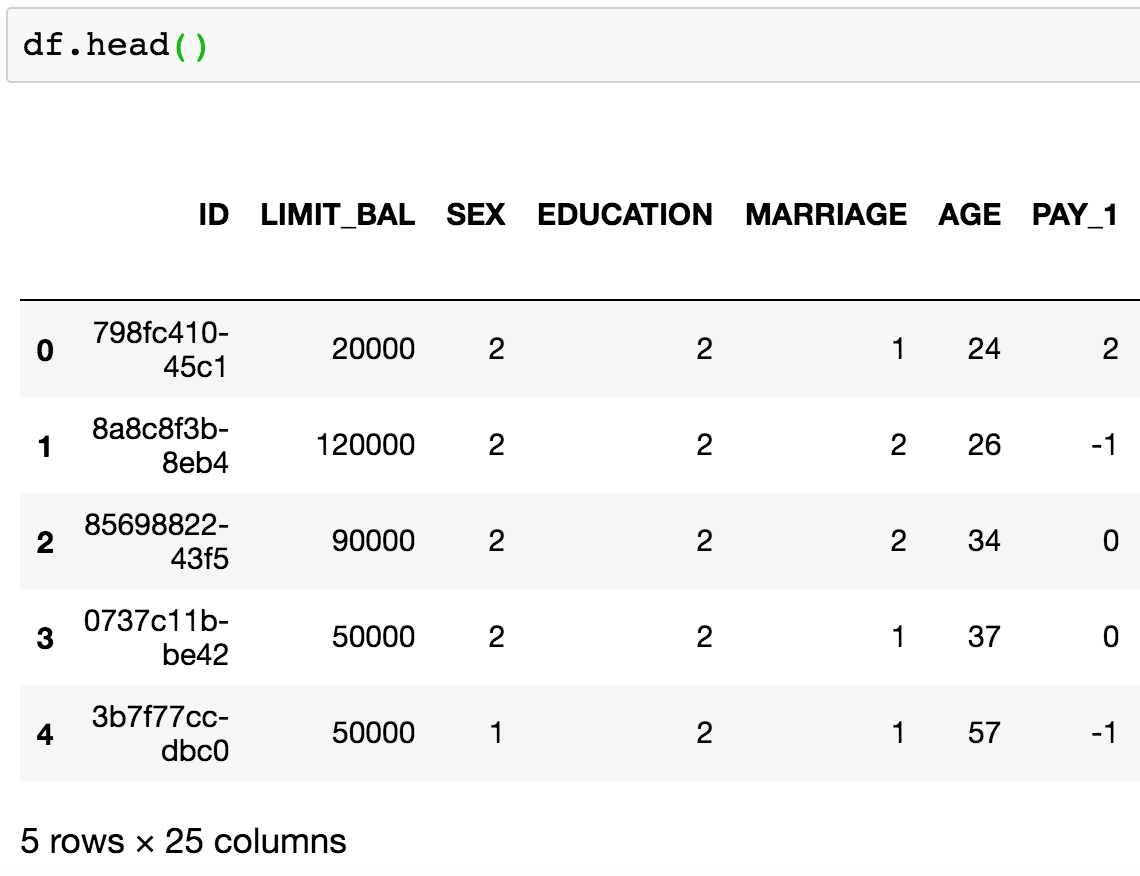Figure 1.19: .head() of a DataFrame

The ID column seems like it contains unique identifiers. Now, to verify if they are in fact unique throughout the whole dataset, we can count the number of unique values using the .nunique() method on the Series (aka column) ID. We first select the column using square brackets.

3. Select the target column (ID) and count unique values using the following command:

df['ID'].nunique()

You will see in the following output that the number of unique entries is 29,687: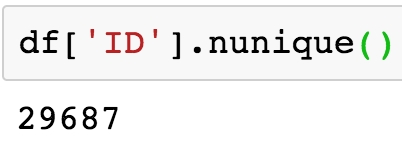Figure 1.20: Finding a data quality issue

4. Run the following command to obtain the number of rows in the dataset:

df.shape

As can be observed in the following output, the total number of rows in the dataset is 30,000: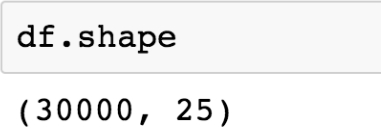Figure 1.21: Dimensions of the dataset

We see here that the number of unique IDs is less than the number of rows. This implies that the ID is not a unique identifier for the rows of the data, as we thought. So we know that there is some duplication of IDs. But how much? Is one ID duplicated many times? How many IDs are duplicated?

We can use the .value_counts() method on the ID series to start to answer these questions. This is similar to a group by/count procedure in SQL. It will list the unique IDs and how often they occur. We will perform this operation in the next step and store the value counts in a variable id_counts.

5. Store the value counts in a variable defined as id_counts and then display the stored values using the .head() method, as shown:

id_counts = df['ID'].value_counts()

You will obtain the following output: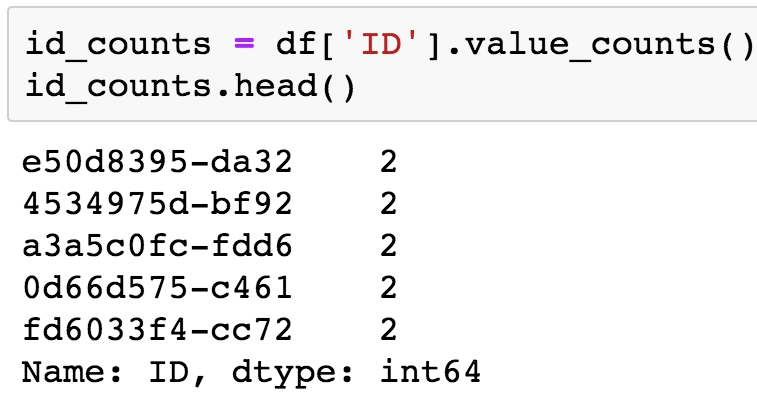Figure 1.22: Getting value counts of the account IDs

Note that .head() returns the first five rows by default. You can specify the number of items to be displayed by passing the required number in the parentheses, ().

6. Display the number of grouped duplicated entries by running another value count:

id_counts.value_counts()

You will obtain the following output: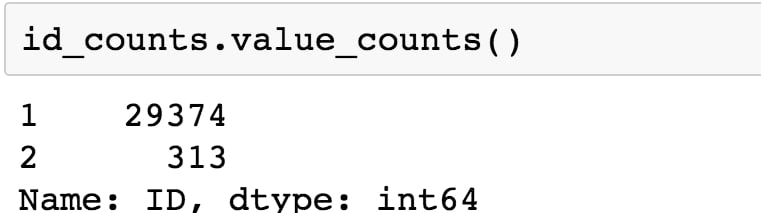Figure 1.23: Getting value counts of the account IDs

In the preceding output and from the initial value count, we can see that most IDs occur exactly once, as expected. However, 313 IDs occur twice. So, no ID occurs more than twice. Armed with this information, we are ready to begin taking a closer look at this data quality issue and fixing it. We will be creating Boolean masks to further clean the data.

To help clean the case study data, we introduce the concept of a logical mask, also known as a Boolean mask. A logical mask is a way to filter an array, or series, by some condition. For example, we can use the "is equal to" operator in Python, ==, to find all locations of an array that contain a certain value. Other comparisons, such as "greater than" (>), "less than" (<), "greater than or equal to" (>=), and "less than or equal to" (<=), can be used similarly. The output of such a comparison is an array or series of True/False values, also known as Boolean values. Each element of the output corresponds to an element of the input, is True if the condition is met, and is False otherwise. To illustrate how this works, we will use synthetic data. Synthetic data is data that is created to explore or illustrate a concept. First, we are going to import the NumPy package, which has many capabilities for generating random numbers, and give it the alias np:

import numpy as np

Now we use what's called a seed for the random number generator. If you set the seed, you will get the same results from the random number generator across runs. Otherwise this is not guaranteed. This can be a helpful option if you use random numbers in some way in your work and want to have consistent results every time you run a notebook:

np.random.seed(seed=24)

Next, we generate 100 random integers, chosen from between 1 and 5 (inclusive). For this we can use numpy.random.randint, with the appropriate arguments.

random_integers = np.random.randint(low=1,high=5,size=100)

Let's look at the first five elements of this array, with random_integers[:5]. The output should appear as follows: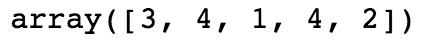Figure 1.24: Random integers

Suppose we wanted to know the locations of all elements of random_integers equal to 3. We could create a Boolean mask to do this.

is_equal_to_3 = random_integers == 3

From examining the first 5 elements, we know the first element is equal to 3, but none of the rest are. So in our Boolean mask, we expect True in the first position and False in the next 4 positions. Is this the case?

is_equal_to_3[:5]

The preceding code should give this output: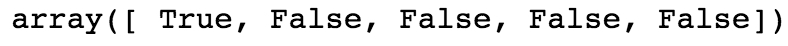Figure 1.25: Boolean mask for the random integers

This is what we expected. This shows the creation of a Boolean mask. But what else can we do with them? Suppose we wanted to know how many elements were equal to 3. To know this, you can take the sum of a Boolean mask, which interprets True as 1 and False as 0:

sum(is_equal_to_3)

This should give us the following output: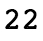Figure 1.26: Sum of the Boolean mask

This makes sense, as with a random, equally likely choice of 5 possible values, we would expect each value to appear about 20% of the time. In addition to seeing how many values in the array meet the Boolean condition, we can also use the Boolean mask to select the elements of the original array that meet that condition. Boolean masks can be used directly to index arrays, as shown here:

random_integers[is_equal_to_3]

This outputs the elements of random_integers meeting the Boolean condition we specified. In this case, the 22 elements equal to 3: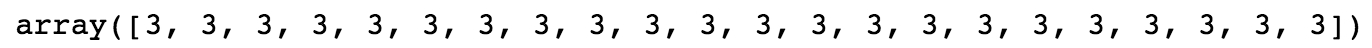Figure 1.27: Using the Boolean mask to index an array

Now you know the basics of Boolean arrays, which are useful in many situations. In particular, you can use the .loc method of DataFrames to index the rows of the DataFrames by a Boolean mask, and the columns by label. Let's continue exploring the case study data with these skills.

Exercise 4: Continuing Verification of Data Integrity

In this exercise, with our knowledge of Boolean arrays, we will examine some of the duplicate IDs we discovered. In Exercise 3, we learned that no ID appears more than twice. We can use this learning to locate the duplicate IDs and examine them. Then we take action to remove rows of dubious quality from the dataset. Perform the following steps to complete the exercise:

Note

The code and the output graphics for this exercise have been loaded in a Jupyter Notebook that can be found here: http://bit.ly/2W9cwPH.

1. Continuing where we left off in Exercise 3, we want the indices of the id_counts series, where the count is 2, to locate the duplicates. We assign the indices of the duplicated IDs to a variable called dupe_mask and display the first 5 duplicated IDs using the following commands:

You will obtain the following output: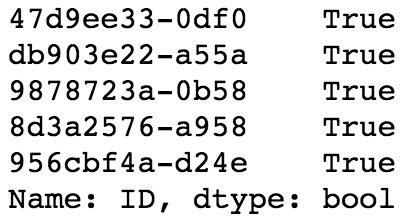Figure 1.28: A Boolean mask to locate duplicate IDs

Here, dupe_mask is the logical mask that we have created for storing the Boolean values.

Note that in the preceding output, we are displaying only the first five entries using dupe_mask to illustrate to contents of this array. As always, you can edit the indices in the square brackets ([]) to change the number of entries displayed.

Our next step is to use this logical mask to select the IDs that are duplicated. The IDs themselves are contained as the index of the id_count series. We can access the index in order to use our logical mask for selection purposes.

2. Access the index of id_count and display the first five rows as context using the following command:

id_counts.index[0:5]

With this, you will obtain the following output: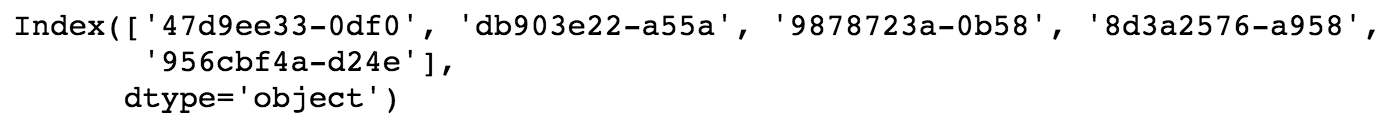Figure 1.29: Duplicated IDs

3. Select and store the duplicated IDs in a new variable called dupe_ids using the following command:

4. Convert dupe_ids to a list and then obtain the length of the list using the following commands:

dupe_ids = list(dupe_ids)
len(dupe_ids)

You should obtain the following output: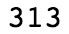Figure 1.30: Output displaying the list length

We changed the dupe_ids variable to a list, as we will need it in this form for future steps. The list has a length of 313, as can be seen in the preceding output, which matches our knowledge of the number of duplicate IDs from the value count.

5. We verify the data in dupe_ids by displaying the first five entries using the following command:

dupe_ids[0:5]

We obtain the following output: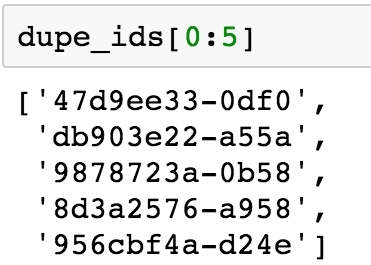Figure 1.31: Making a list of duplicate IDs

We can observe from the preceding output that the list contains the required entries of duplicate IDs. We're now in a position to examine the data for the IDs in our list of duplicates. In particular, we'd like to look at the values of the features, to see what, if anything, might be different between these duplicate entries. We will use the .isin and .loc methods for this purpose.

Using the first three IDs on our list of dupes, dupe_ids[0:3], we will plan to first find the rows containing these IDs. If we pass this list of IDs to the .isin method of the ID series, this will create another logical mask we can use on the larger DataFrame to display the rows that have these IDs. The .isin method is nested in a .loc statement indexing the DataFrame in order to select the location of all rows containing "True" in the Boolean mask. The second argument of the .loc indexing statement is :, which implies that all columns will be selected. By performing the following steps, we are essentially filtering the DataFrame in order to view all the columns for the first three duplicate IDs.

6. Run the following command in your Notebook to execute the plan we formulated in the previous step: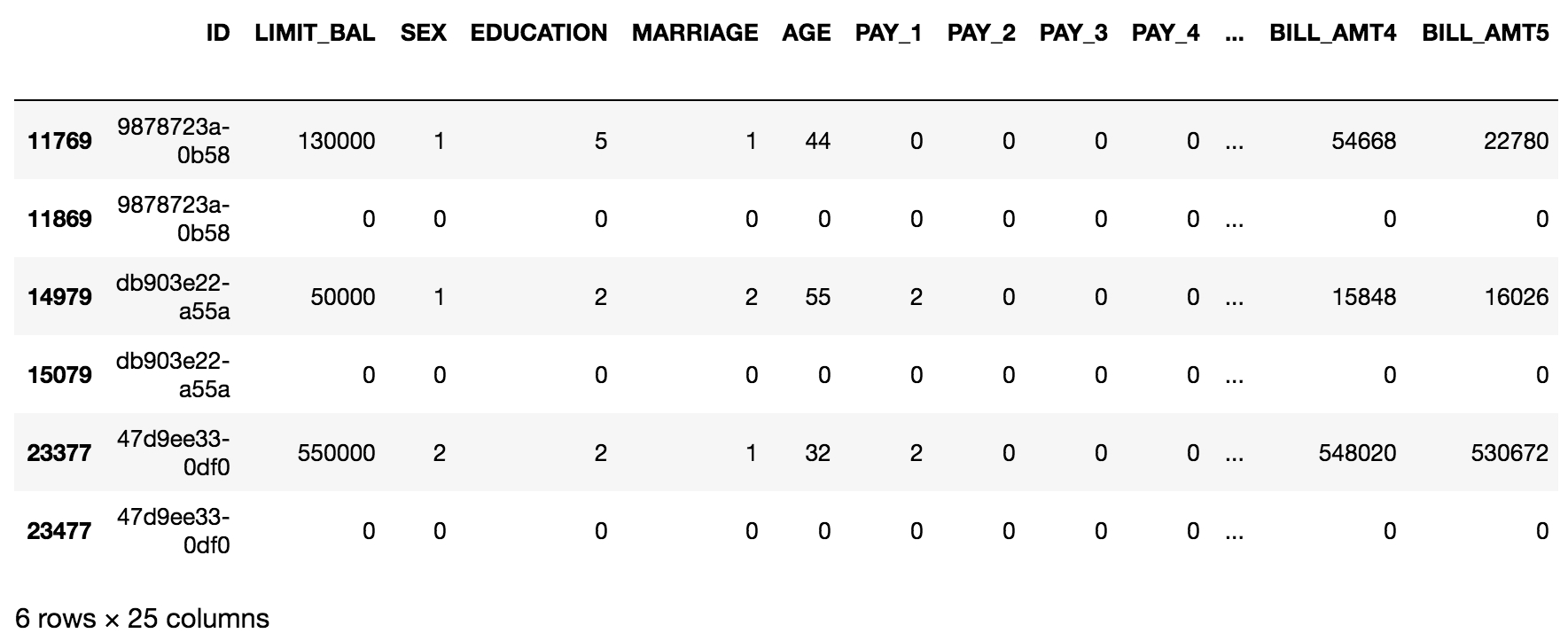Figure 1.32: Examining the data for duplicate IDs

What we observe here is that each duplicate ID appears to have one row with what seems like valid data, and one row of entirely zeros. Take a moment and think to yourself what you would do with this knowledge.

After some reflection, it should be clear that you ought to delete the rows with all zeros. Perhaps these arose through a faulty join condition in the SQL query that generated the data? Regardless, a row of all zeros is definitely invalid data as it makes no sense for someone to have an age of 0, a credit limit of 0, and so on.

One approach to deal with this issue would be to find rows that have all zeros, except for the first column, which has the IDs. These would be invalid data in any case, and it may be that if we get rid of all of these, we would also solve our problem of duplicate IDs. We can find the entries of the DataFrame that are equal to zero by creating a Boolean matrix that is the same size as the whole DataFrame, based on the "is equal to zero" condition.

7. Create a Boolean matrix of the same size as the entire DataFrame using ==, as shown:

In the next steps, we'll use df_zero_mask, which is another DataFrame containing Boolean values. The goal will be to create a Boolean series, feature_zero_mask, that identifies every row where all the elements starting from the second column (the features and response, but not the IDs) are 0. To do so, we first need to index df_zero_mask using the integer indexing (.iloc) method. In this method, we pass (:) to examine all rows and (1:) to examine all columns starting with the second one (index 1). Finally, we will apply the all() method along the column axis (axis=1), which will return True if and only if every column in that row is True. This is a lot to think about, but it's pretty simple to code, as will be observed in the following step.

8. Create the Boolean series feature_zero_mask, as shown in the following:

9. Calculate the sum of the Boolean series using the following command:

You should obtain the following output: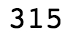Figure 1.33: The number of rows with all zeros except for the ID

The preceding output tells us that 315 rows have zeros for every column but the first one. This is greater than the number of duplicate IDs (313), so if we delete all the "zero rows," we may get rid of the duplicate ID problem.

10. Clean the DataFrame by eliminating the rows with all zeros, except for the ID, using the following code:

While performing the cleaning operation in the preceding step, we return a new DataFrame called df_clean_1. Notice that here we've used the .copy() method after the .loc indexing operation to create a copy of this output, as opposed to a view on the original DataFrame. You can think of this as creating a new DataFrame, as opposed to referencing the original one. Within the .loc method, we used the logical not operator, ~, to select all the rows that don't have zeros for all the features and response, and : to select all columns. These are the valid data we wish to keep. After doing this, we now want to know if the number of remaining rows is equal to the number of unique IDs.

11. Verify the number of rows and columns in df_clean_1 by running the following code:

df_clean_1.shape

You will obtain the following output: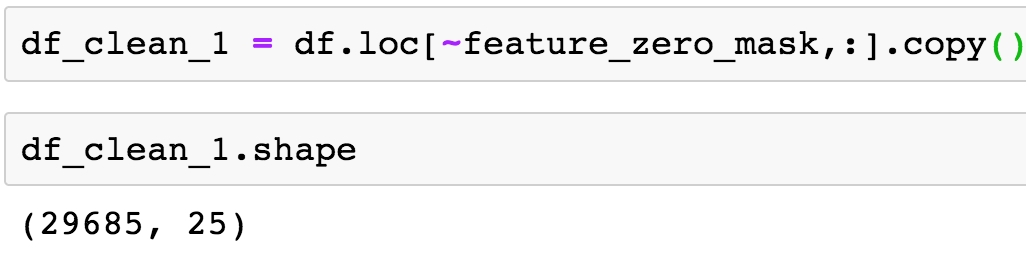Figure 1.34: Dimensions of the cleaned DataFrame

12. Obtain the number of unique IDs by running the following code:

df_clean_1['ID'].nunique()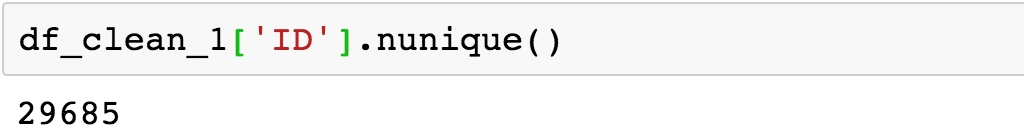Figure 1.35: Number of unique IDs in the cleaned DataFrame

From the preceding output, we can see that we have successfully eliminated duplicates, as the number of unique IDs is equal to the number of rows. Now take a breath and pat yourself on the back. That was a whirlwind introduction to quite a few pandas techniques for indexing and characterizing data. Now that we've filtered out the duplicate IDs, we're in a position to start looking at the actual data itself: the features, and eventually, the response. We'll walk you through this process.

Exercise 5: Exploring and Cleaning the Data

Thus far, we have identified a data quality issue related to the metadata: we had been told that every sample from our dataset corresponded to a unique account ID, but found that this was not the case. We were able to use logical indexing and pandas to correct this issue. This was a fundamental data quality issue, having to do simply with what samples were present, based on the metadata. Aside from this, we are not really interested in the metadata column of account IDs: for the time being these will not help us develop a predictive model for credit default.

Now, we are ready to start examining the values of the features and response, the data we will use to develop our predictive model. Perform the following steps to complete this exercise:

Note

The code and the resulting output for this exercise have been loaded in a Jupyter Notebook that can be found here: http://bit.ly/2W9cwPH.

• -2 means the account started that month with a zero balance, and never used any credit

• -1 means the account had a balance that was paid in full

• 0 means that at least the minimum payment was made, but the entire balance wasn't paid (that is, a positive balance was carried to the next month)

We thank our business partner since this answers our questions, for now. Maintaining a good line of communication and working relationship with the business partner is important, as you can see here, and may determine the success or failure of a project.

1. Obtain the data type of the columns in the data by using the .info() method as shown:

df_clean_1.info()

You should see the following output: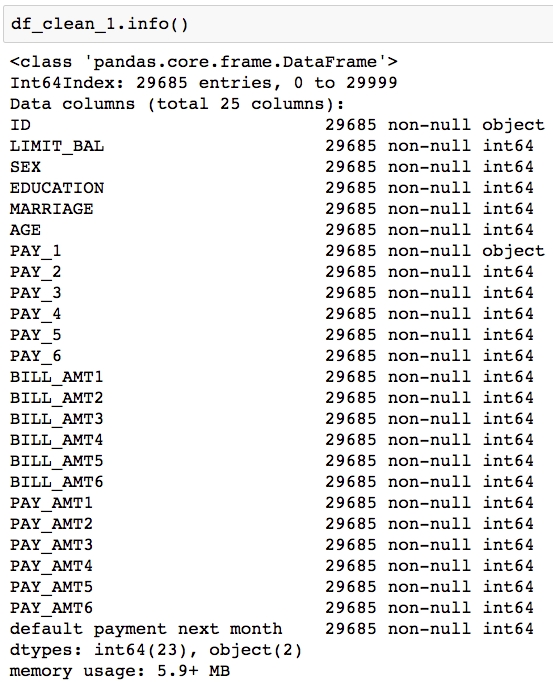We can see in Figure 1.34 that there are 25 columns. Each row has 29,685 non-null values, according to this summary, which is the number of rows in the DataFrame. This would indicate that there is no missing data, in the sense that each cell contains some value. However, if there is a fill value to represent missing data, that would not be evident here.

We also see that most columns say int64 next to them, indicating they are an integer data type, that is, numbers such as ..., -2, -1, 0, 1, 2,... . The exceptions are ID and PAY_1. We are already familiar with ID; this contains strings, which are account IDs. What about PAY_1? According to the values in the data dictionary, we'd expect this to contain integers, like all the other features. Let's take a closer look at this column.

2. Use the.head(n) pandas method to view the top n rows of the PAY_1 series:

You should obtain the following output: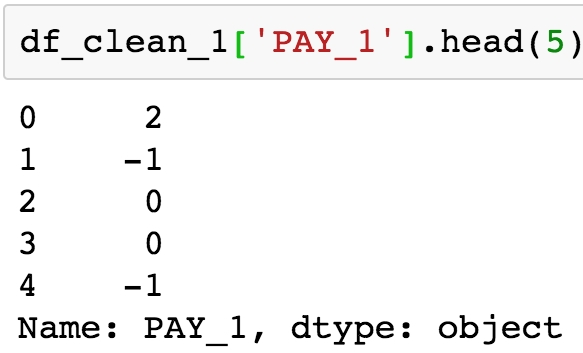Figure 1.37: Examine a few columns' contents

The integers on the left of the output are the index, which are simply consecutive integers starting with 0. The data from the PAY_1 column is shown on the left. This is supposed to be the payment status of the most recent month's bill, using values –1, 1, 2, 3, and so on. However, we can see that there are values of 0 here, which are not documented in the data dictionary. According to the data dictionary, "The measurement scale for the repayment status is: -1 = pay duly; 1 = payment delay for one month; 2 = payment delay for two months; . . .; 8 = payment delay for eight months; 9 = payment delay for nine months and above" (https://archive.ics.uci.edu/ml/datasets/default+of+credit+card+clients). Let's take a closer look, using the value counts of this column.

3. Obtain the value counts for the PAY_1 column by using .value_counts() method:

df_clean1['PAY_1'].value_counts()

You should see the following output: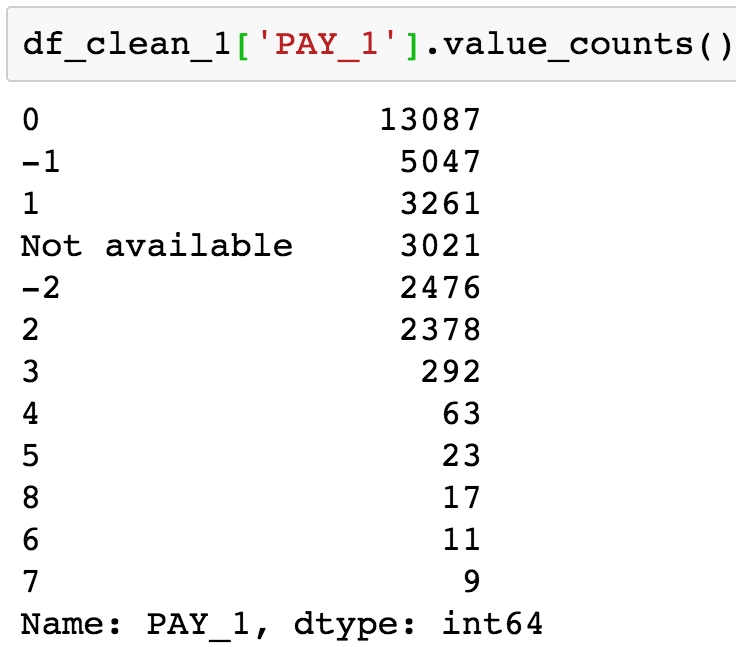Figure 1.38: Value counts of the PAY_1 column

The preceding output reveals the presence of two undocumented values: 0 and –2, as well as the reason this column was imported by pandas as an object data type, instead of int64 as we would expect for integer data. There is a 'Not available' string present in this column, symbolizing missing data. Later on in the book, we'll come back to this when we consider how to deal with missing data. For now, we'll remove rows of the dataset, for which this feature has a missing value.

4. Use a logical mask with the != operator (which means "does not equal" in Python) to find all the rows that don't have missing data for the PAY_1 feature:

valid_pay_1_mask = df_clean_1['PAY_1'] != 'Not available'

By running the preceding code, you will obtain the following output: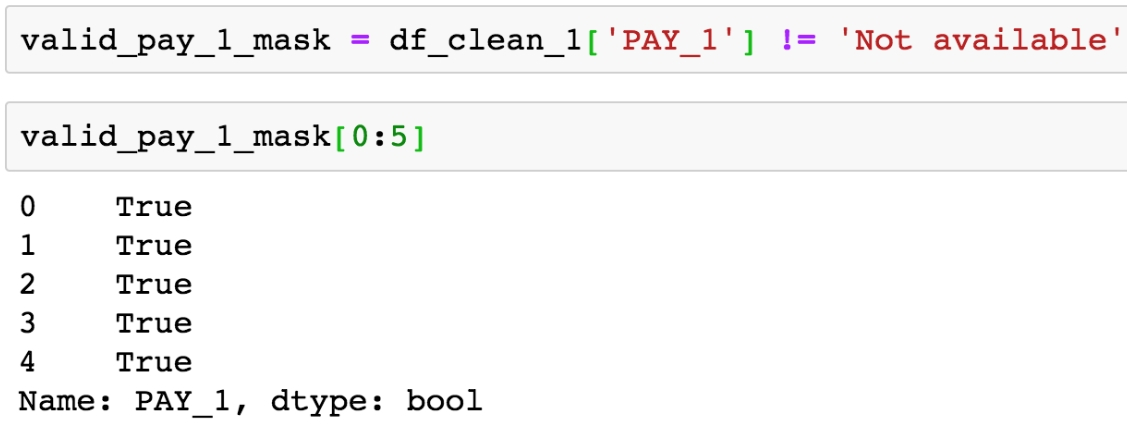Figure 1.39: Creating a Boolean mask

5. Check how many rows have no missing data by calculating the sum of the mask:

You will obtain the following output: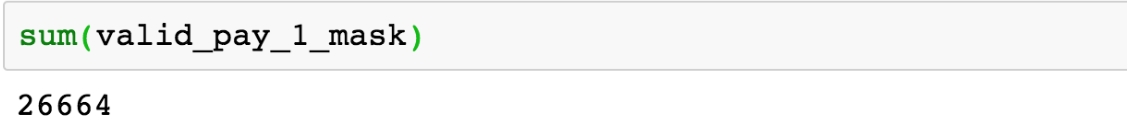Figure 1.40: Sum of the Boolean mask for non-missing data

We see that 26,664 rows do not have the value 'Not available' in the PAY_1 column. We saw from the value count that 3,021 rows do have this value, and 29,685 – 3,021 = 26,664, so this checks out.

6. Clean the data by eliminating the rows with the missing values of PAY_1 as shown:

7. Obtain the shape of the cleaned data using the following command:

df_clean_2.shape

You will obtain the following output: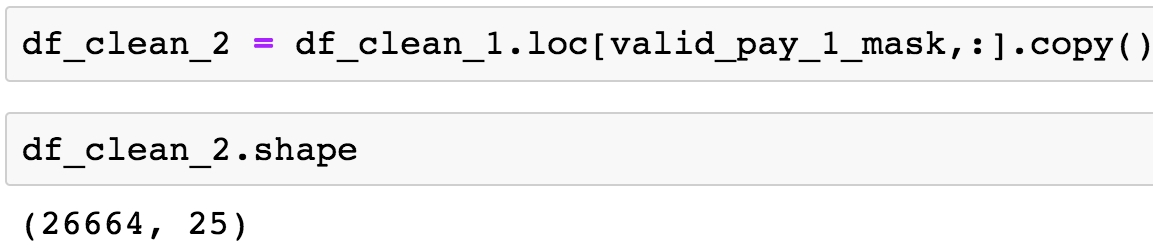Figure 1.41: Shape of the cleaned data

After removing these rows, we check that the resulting DataFrame has the expected shape. You can also check for yourself whether the value counts indicate the desired values have been removed like this: df_clean_2['PAY_1'].value_counts().

Lastly, so this column's data type can be consistent with the others, we will cast it from the generic object type to int64 like all the other features, using the .astype method. Then we select a couple columns, including PAY_1, to examine the data types and make sure it worked.

8. Run the following command to convert the data type for PAY_1 from object to int64 and show the column metadata for PAY_1 and PAY_2:

df_clean_2['PAY_1'] = df_clean_2['PAY_1'].astype('int64')
df_clean_2[['PAY_1', 'PAY_2']].info()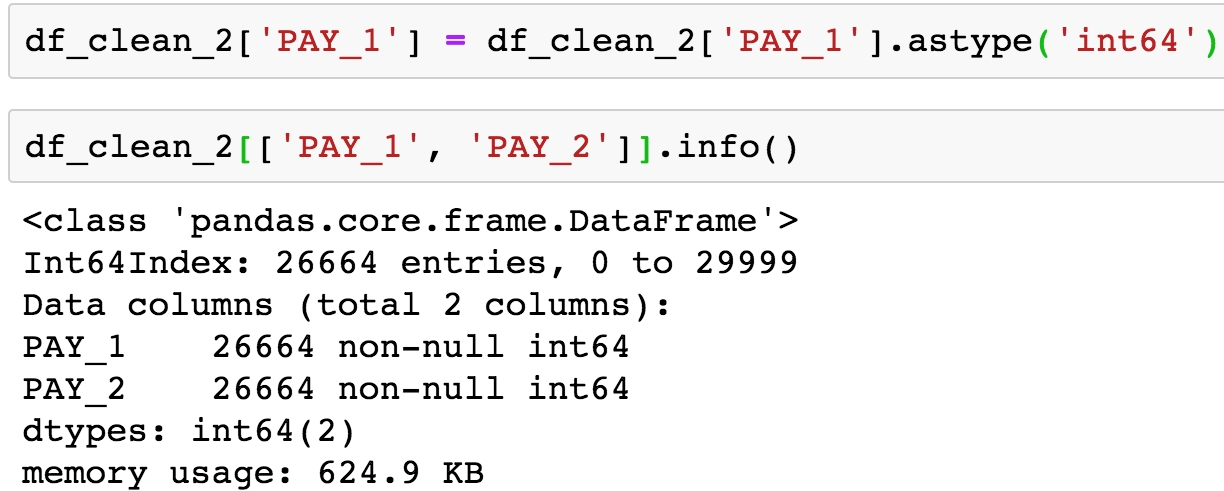Figure 1.42: Check the data type of the cleaned column

Congratulations, you have completed your second data cleaning operation! However, if you recall, during this process we also noticed the undocumented values of –2 and 0 in PAY_1. Now, let's imagine we got back in touch with our business partner and learned the following information:

Data Quality Assurance and Exploration

So far, we remedied two data quality issues just by asking basic questions or by looking at the .info() summary. Let's now take a look at the first few columns. Before we get to the historical bill payments, we have the credit limits of the accounts of LIMIT_BAL, and the demographic features SEX, EDUCATION, MARRIAGE, and AGE. Our business partner has reached out to us, to let us know that gender should not be used to predict credit-worthiness, as this is unethical by their standards. So we keep this in mind for future reference. Now we explore the rest of these columns, making any corrections that are necessary.

In order to further explore the data, we will use histograms. Histograms are a good way to visualize data that is on a continuous scale, such as currency amounts and ages. A histogram groups similar values into bins, and shows the number of data points in these bins as a bar graph.

To plot histograms, we will start to get familiar with the graphical capabilities of pandas. pandas relies on another library called Matplotlib to create graphics, so we'll also set some options using matplotlib. Using these tools, we'll also learn how to get quick statistical summaries of data in pandas.

Exercise 6: Exploring the Credit Limit and Demographic Features

In this exercise, we start our exploration of data with the credit limit and age features. We will visualize them and get summary statistics to check that the data contained in these features is sensible. Then we will look at the education and marriage categorical features to see if the values there make sense, and correct them as necessary. LIMIT_BAL and AGE are numerical features, meaning they are measured on a continuous scale. Consequently, we'll use histograms to visualize them. Perform the following steps to complete the exercise:

Note

The code and the resulting output for this exercise have been loaded in a Jupyter Notebook that can found here: http://bit.ly/2W9cwPH.

1. Import matplotlib and set up some plotting options with this code snippet:

import matplotlib.pyplot as plt #import plotting package

#render plotting automatically
%matplotlib inline

import matplotlib as mpl #additional plotting functionality

mpl.rcParams['figure.dpi'] = 400 #high resolution figures

This imports matplotlib and uses .rcParams to set the resolution (dpi = dots per inch) for a nice crisp image; you may not want to worry about this last part unless you are preparing things for presentation, as it could make the images quite large in your notebook.

2. Run df_clean_2[['LIMIT_BAL', 'AGE']].hist() and you should see the following histograms: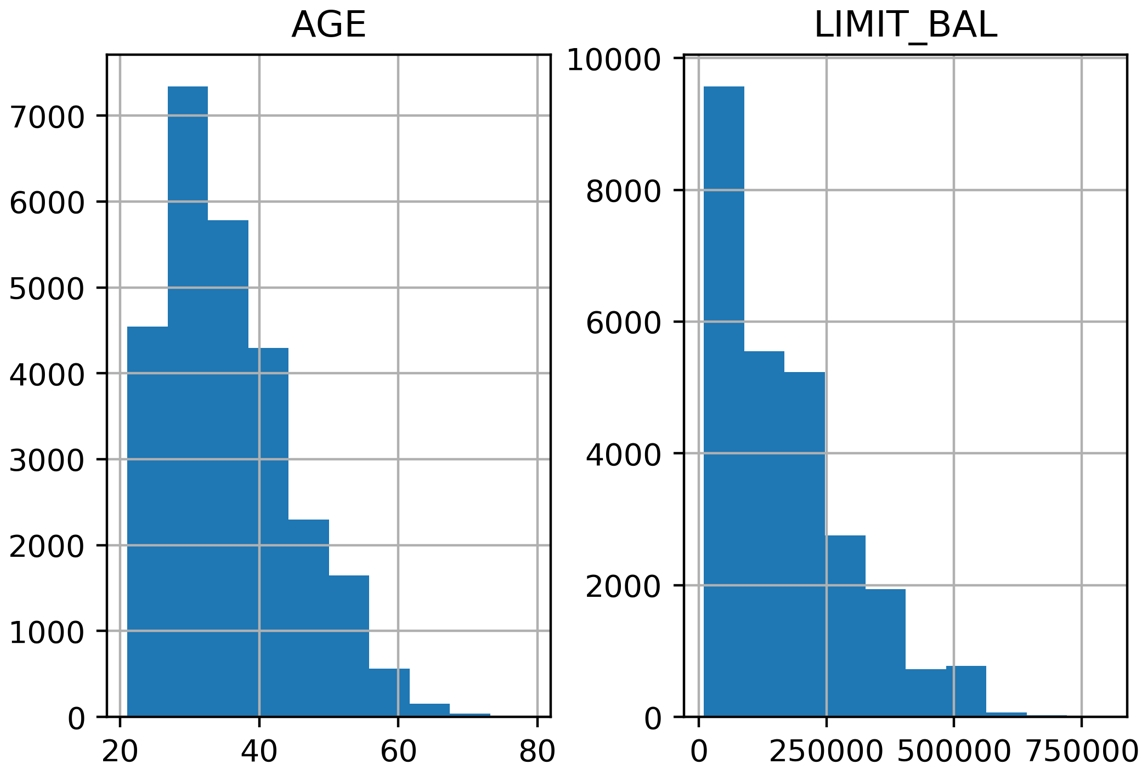Figure 1.43: Histograms of the credit limit and age data

This is nice visual snapshot of these features. We can get a quick, approximate look at all of the data in this way. In order to see statistics such as mean and median (that is, the 50th percentile), there is another helpful pandas function.

3. Generate a tabular report of summary statistics using the following command:

df_clean_2[['LIMIT_BAL', 'AGE']].describe()

You should see the following output: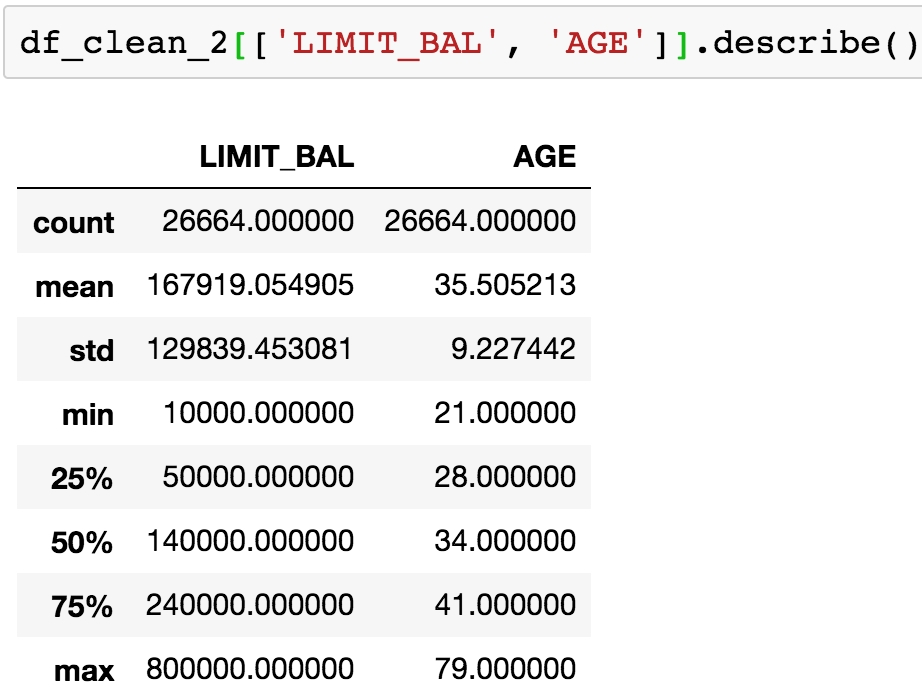Figure 1.44: Statistical summaries of credit limit and age data

Based on the histograms and the convenient statistics computed by .describe(), which include a count of non-nulls, the mean and standard deviation, minimum, maximum, and quartiles, we can make a few judgements.

LIMIT_BAL, the credit limit, seems to make sense. The credit limits have a minimum of 10,000. This dataset is from Taiwan; the exact unit of currency (NT dollar) may not be familiar, but intuitively, a credit limit should be above zero. You are encouraged to look up the conversion to your local currency and consider these credit limits. For example, 1 US dollar is about 30 NT dollars.

The AGE feature also looks reasonably distributed, with no one under the age of 21 having a credit account.

For the categorical features, a look at the value counts is useful, since there are relatively few unique values.

4. Obtain the value counts for the EDUCATION feature using the following code:

df_clean_2['EDUCATION'].value_counts()

You should see this output: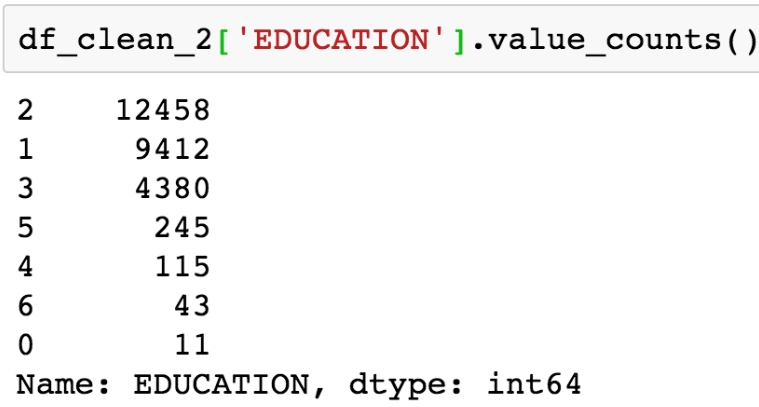Figure 1.45: Value counts of the EDUCATION feature

Here, we see undocumented education levels 0, 5, and 6, as the data dictionary describes only "Education (1 = graduate school; 2 = university; 3 = high school; 4 = others)". Our business partner tells us they don't know about the others. Since they are not very prevalent, we will lump them in with the "others" category, which seems appropriate, with our client's blessing, of course.

5. Run this code to combine the undocumented levels of the EDUCATION feature into the level for "others" and then examine the results:

df_clean_2['EDUCATION'].replace(to_replace=[0, 5, 6], value=4, inplace=True)
df_clean_2['EDUCATION'].value_counts()

The pandas .replace method makes doing the replacements described in the preceding step pretty quick. Once you run the code, you should see this output: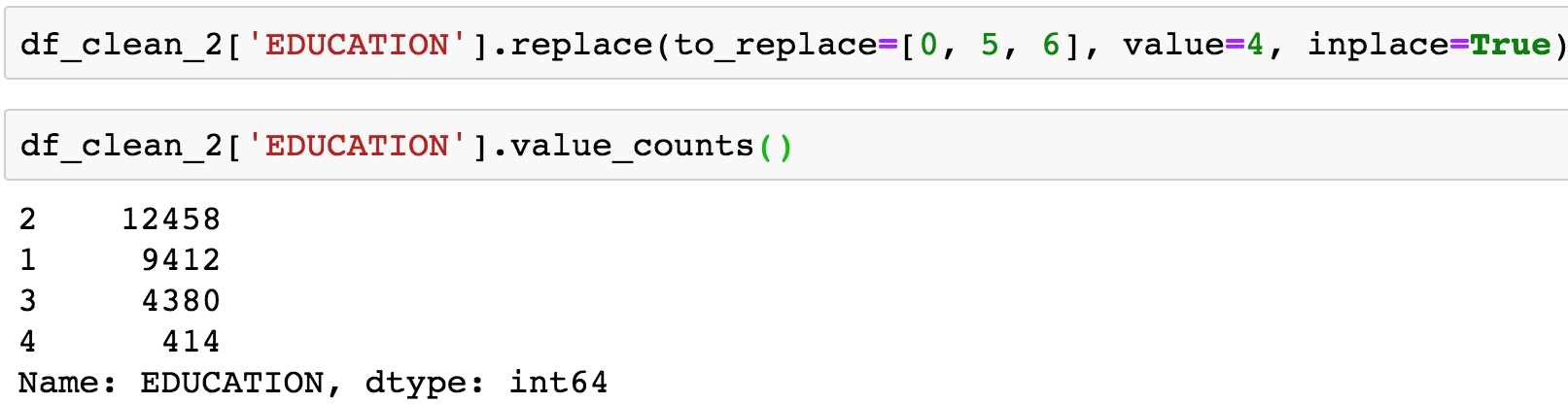Figure 1.46: Cleaning the EDUCATION feature

Note that here we make this change in place (inplace=True). This means that, instead of returning a new DataFrame, this operation will make the change on the existing DataFrame.

6. Obtain the value counts for the MARRIAGE feature using the following code:

df_clean_2['MARRIAGE'].value_counts()

You should obtain the following output: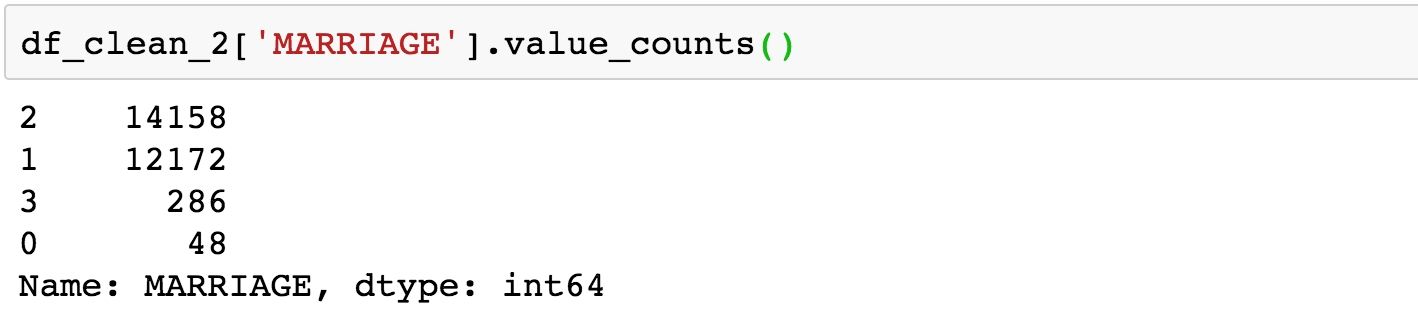Figure 1.47: Value counts of raw MARRIAGE feature

The issue here is similar to that encountered for the EDUCATION feature; there is a value, 0, which is not documented in the data dictionary: "1 = married; 2 = single; 3 = others". So we'll lump it in with "others".

7. Change the values of 0 in the MARRIAGE feature to 3 and examine the result with this code:

df_clean_2['MARRIAGE'].replace(to_replace=0, value=3, inplace=True)
df_clean_2['MARRIAGE'].value_counts()

The output should be: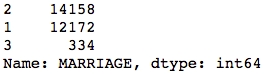Figure 1.48: Value counts of cleaned MARRIAGE feature

We've now accomplished a lot of exploration and cleaning of the data. We will do some more advanced visualization and exploration of the financial history features, that come after this in the DataFrame, later.

Deep Dive: Categorical Features

Machine learning algorithms only work with numbers. If your data contains text features, for example, these would require transformation to numbers in some way. We learned above that the data for our case study is, in fact, entirely numerical. However, it's worth thinking about how it got to be that way. In particular, consider the EDUCATION feature.

This is an example of what is called a categorical feature: you can imagine that as raw data, this column consisted of the text labels 'graduate school', 'university', 'high school', and 'others'. These are called the levels of the categorical feature; here, there are four levels. It is only through a mapping, which has already been chosen for us, that these data exist as the numbers 1, 2, 3, and 4 in our dataset. This particular assignment of categories to numbers creates what is known as an ordinal feature, since the levels are mapped to numbers in order. As a data scientist, at a minimum you need to be aware of such mappings, if you are not choosing them yourself.

What are the implications of this mapping?

It makes some sense that the education levels are ranked, with 1 corresponding to the highest level of education in our dataset, 2 to the next highest, 3 to the next, and 4 presumably including the lowest levels. However, when you use this encoding as a numerical feature in a machine learning model, it will be treated just like any other numerical feature. For some models, this effect may not be desired.

What if a model seeks to find a straight-line relationship between the features and response?

Whether or not this works in practice depends on the actual relationship between different levels of education and the outcome we are trying to predict.

Here, we examine two hypothetical cases of ordinal categorical variables, each with 10 levels. The levels measure the self-reported satisfaction levels from customers visiting a website. The average number of minutes spent on the website for customers reporting each level is plotted on the y-axis. We've also plotted the line of best fit in each case to illustrate how a linear model would deal with these data, as shown in the following figure: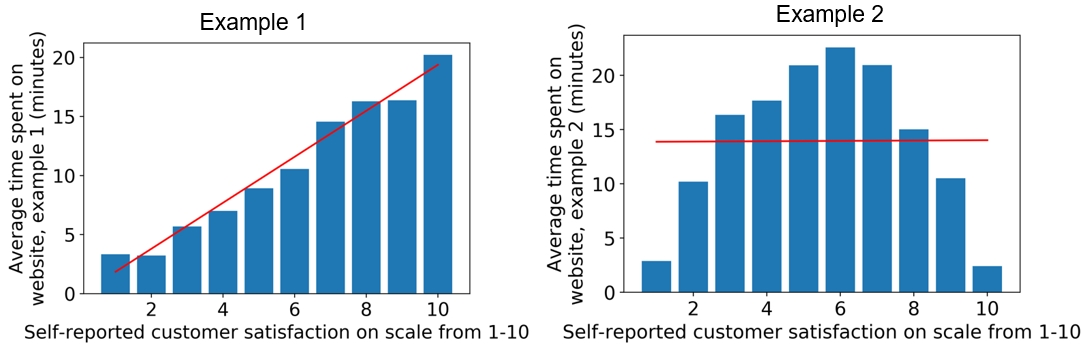Figure 1.49: Ordinal features may or may not work well in a linear model

We can see that if an algorithm assumes a linear (straight-line) relationship between features and response, this may or may not work well depending on the actual relationship between this feature and the response variable. Notice that in the preceding example, we are modeling a regression problem: the response variable takes on a continuous range of numbers. However, some classification algorithms such as logistic regression also assume a linear effect of the features. We will discuss this in greater detail later when we get into modeling the data for our case study.

Roughly speaking, for a binary classification model, you can look at the different levels of a categorical feature in terms of the average values of the response variable, which represent the "rates" of the positive class (i.e., the samples where the response variable = 1) for each level. This can give you an idea of whether an ordinal encoding will work well with a linear model. Assuming you've imported the same packages in your Jupyter notebook as in the previous sections, you can quickly look at this using a groupby/agg and bar plot in pandas:

f_clean_2.groupby('EDUCATION').agg({'default payment next month':'mean'}).plot.bar(legend=False)
plt.ylabel('Default rate')
plt.xlabel('Education level: ordinal encoding')

Once you run the code, you should obtain the following output: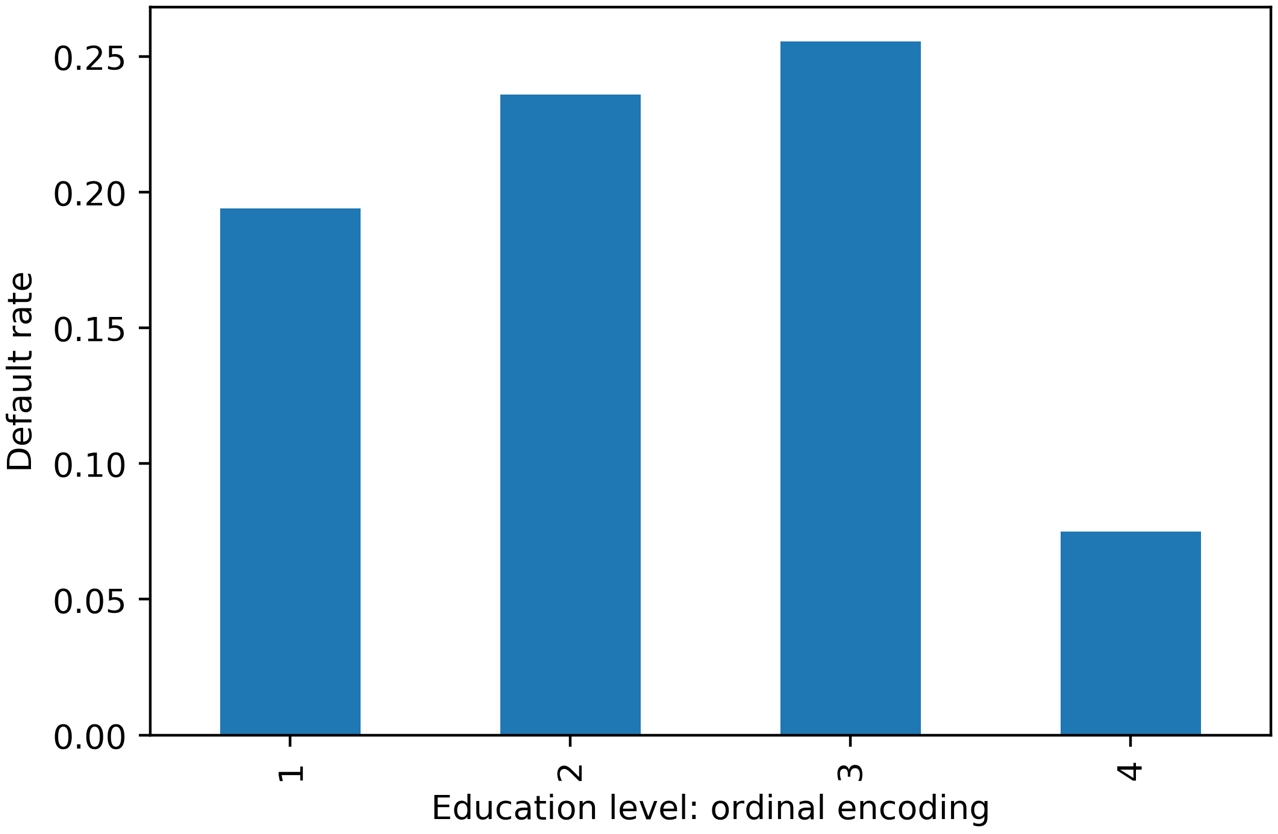Figure 1.50: Default rate within education levels

Similar to example 2 in Figure 1.49, it looks like a straight-line fit would probably not be the best description of the data here. In case a feature has a non-linear effect like this, it may be better to use a more complex algorithm such as a decision tree or random forest. Or, if a simpler and more interpretable linear model such as logistic regression is desired, we could avoid an ordinal encoding and use a different way of encoding categorical variables. A popular way of doing this is called one-hot encoding (OHE).

OHE is a way to transform a categorical feature, which may consist of text labels in the raw data, into a numerical feature that can be used in mathematical models.

Let's learn about this in an exercise. And if you are wondering why a logistic regression is more interpretable and a random forest is more complex, we will be learning about these concepts in detail during the rest of the book.

Note

Categorical variables in different machine learning packages

Some machine learning packages, for instance, certain R packages or newer versions of the Spark platform for big data, will handle categorical variables without assuming they are ordinal. Always be sure to carefully read the documentation to learn what the model will assume about the features, and how to specify whether a variable is categorical, if that option is available.

Exercise 7: Implementing OHE for a Categorical Feature

In this exercise, we will "reverse engineer" the EDUCATION feature in the dataset to obtain the text labels that represent the different education levels, then show how to use pandas to create an OHE.

First, let's consider our EDUCATION feature, before it was encoded as an ordinal. From the data dictionary, we know that 1 = graduate school, 2 = university, 3 = high school, 4 = others. We would like to recreate a column that has these strings, instead of numbers. Perform the following steps to complete the exercise:

Note

The code and the resulting output for this exercise have been loaded in a Jupyter Notebook that can found here: http://bit.ly/2W9cwPH.

1. Create an empty column for the categorical labels called EDUCATION_CAT. Using the following command:

df_clean_2['EDUCATION_CAT'] = 'none'
2. Examine the first few rows of the DataFrame for the EDUCATION and EDUCATION_CAT columns:

The output should appear as follows: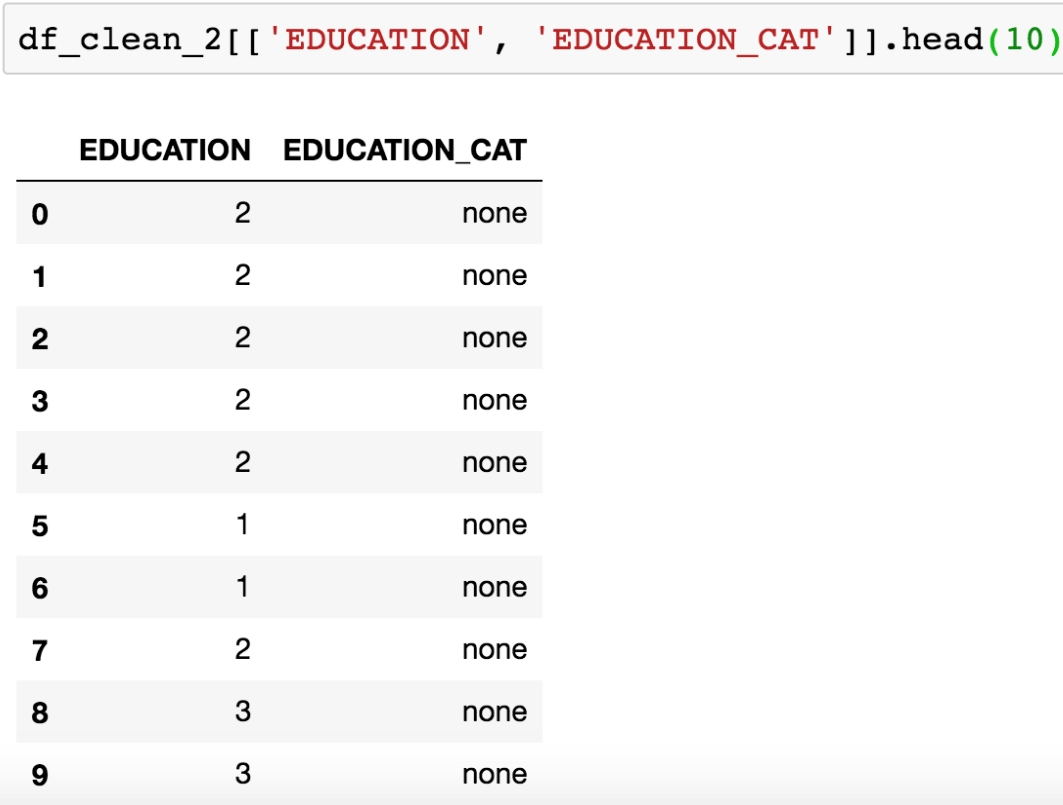Figure 1.51: Selecting columns and viewing the first 10 rows

We need to populate this new column with the appropriate strings. pandas provides a convenient functionality for mapping values of a Series on to new values. This function is in fact called .map and relies on a dictionary to establish the correspondence between the old values and the new values. Our goal here is to map the numbers in EDUCATION on to the strings they represent. For example, where the EDUCATION column equals the number 1, we'll assign the 'graduate school' string to the EDUCATION_CAT column, and so on for the other education levels.

3. Create a dictionary that describes the mapping for education categories using the following code:

cat_mapping = {
2: "university",
3: "high school",
4: "others"
}
4. Apply the mapping to the original EDUCATION column using .map and assign the result to the new EDUCATION_CAT column:

df_clean_2['EDUCATION_CAT'] = df_clean_2['EDUCATION'].map(cat_mapping)

After running those lines, you should see the following output: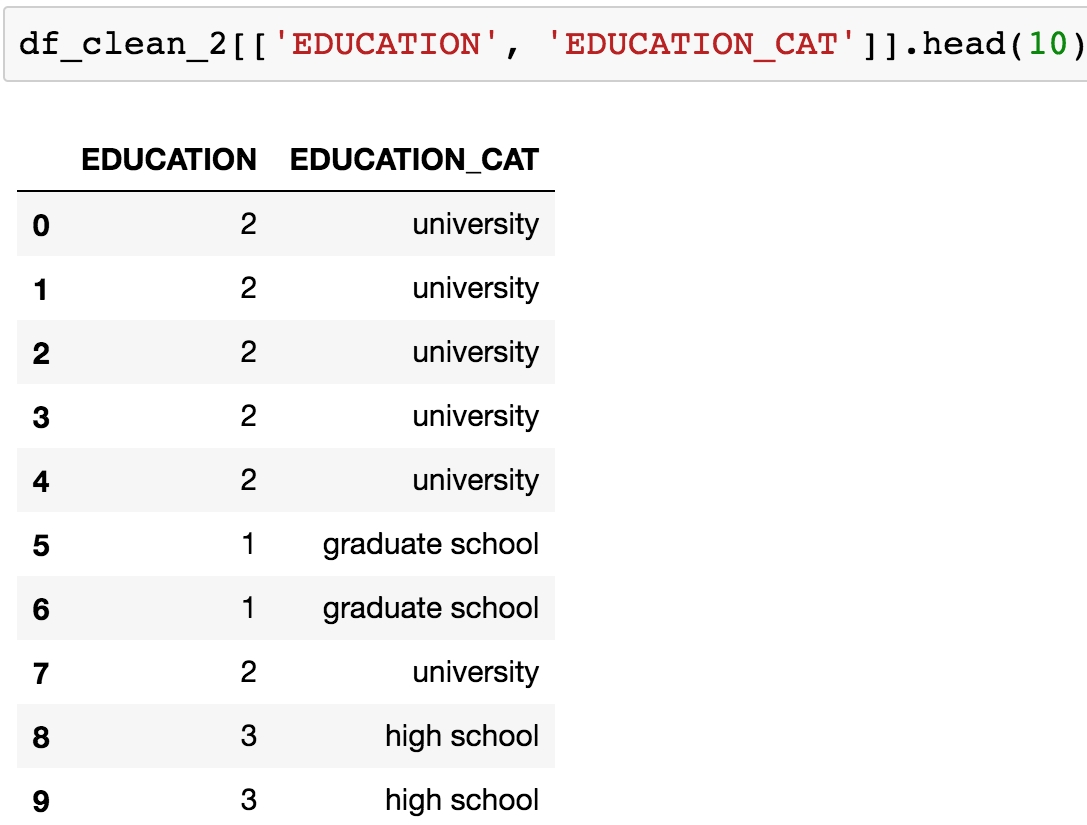Figure 1.52: Examining the string values corresponding to the ordinal encoding of EDUCATION

Excellent! Note that we could have skipped Step 1, where we assigned the new column with 'none', and gone straight to Steps 3 and 4 to create the new column. However, sometimes it's useful to create a new column initialized with a single value, so it's worth knowing how to do that.

Now we are ready to one-hot encode. We can do this by passing a Series of a DataFrame to the pandas get_dummies() function. The function got this name because one-hot encoded columns are also referred to as dummy variables. The result will be a new DataFrame, with as many columns as there are levels of the categorical variable.

5. Run this code to create a one-hot encoded DataFrame of the EDUCATION_CAT column. Examine the first 10 rows:

edu_ohe = pd.get_dummies(df_clean_2['EDUCATION_CAT'])

This should produce the following output: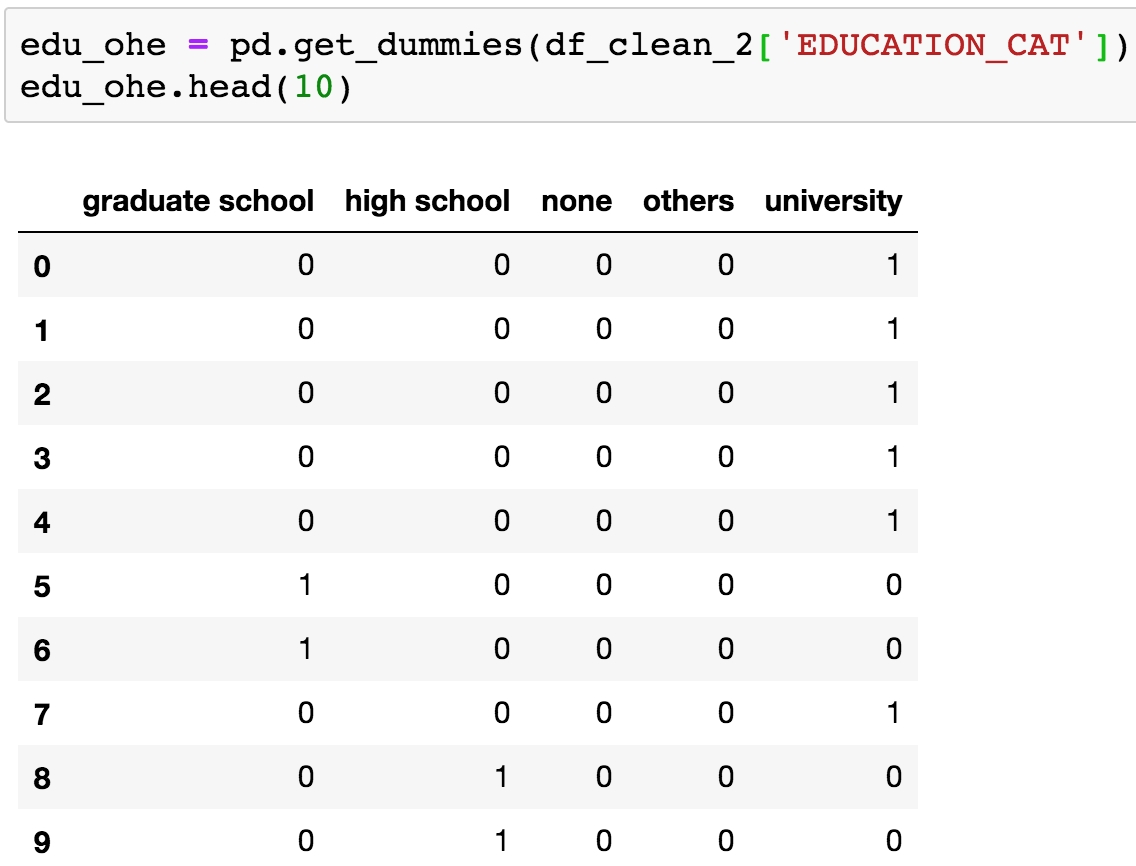Figure 1.53: Data frame of one-hot encoding

You can now see why this is called "one-hot encoding": across all these columns, any particular row will have a 1 in exactly 1 column, and 0s in the rest. For a given row, the column with the 1 should match up to the level of the original categorical variable. To check this, we need to concatenate this new DataFrame with the original one and examine the results side by side. We will use the pandas concat function, to which we pass the list of DataFrames we wish to concatenate, and the axis=1 keyword saying to concatenate them horizontally; that is, along the column axis. This basically means we are combining these two DataFrames "side by side", which we know we can do because we just created this new DataFrame from the original one: we know it will have the same number of rows, which will be in the same order as the original DataFrame.

6. Concatenate the one-hot encoded DataFrame to the original DataFrame as follows:

df_with_ohe = pd.concat([df_clean_2, edu_ohe], axis=1)

You should see this output: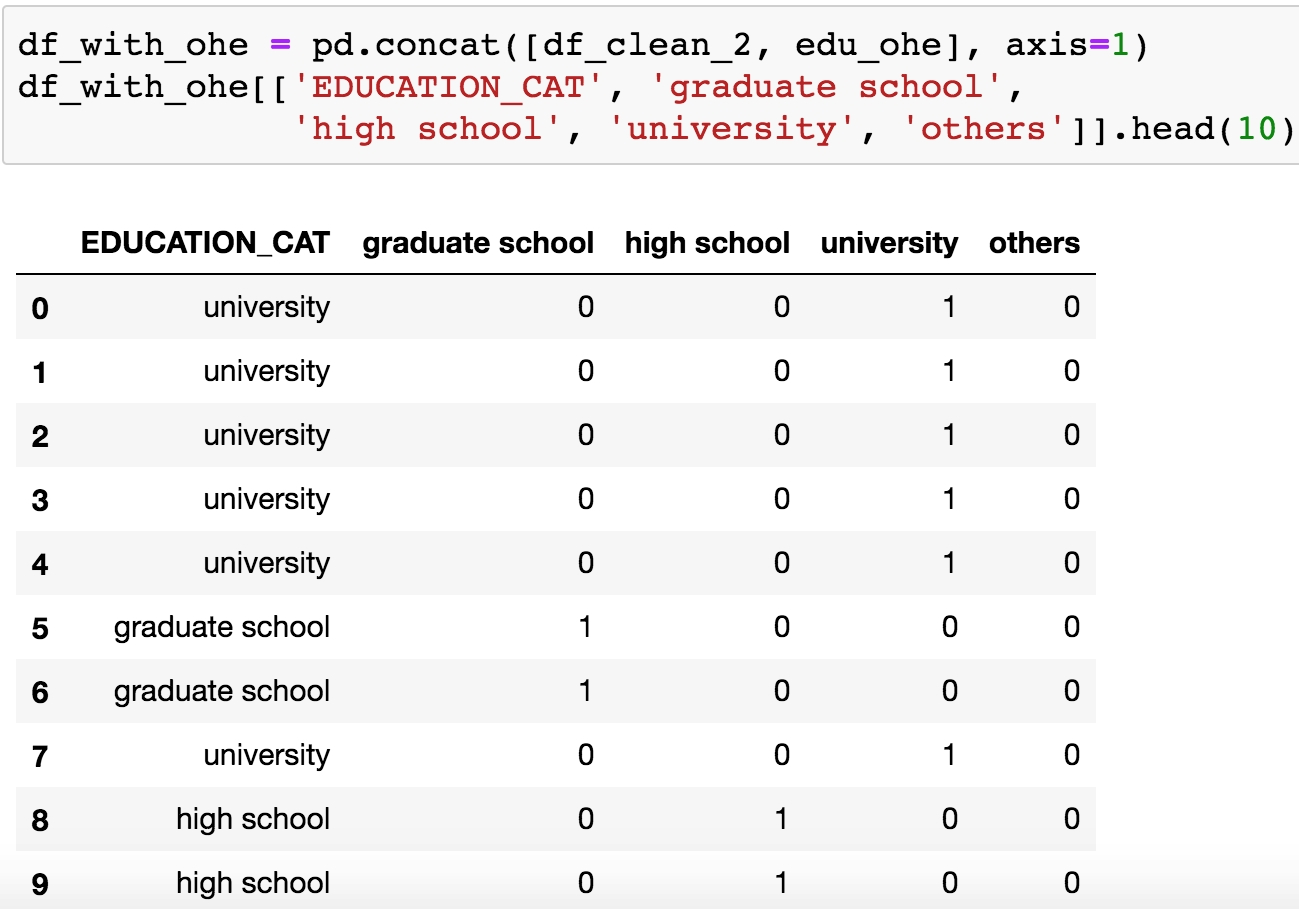Figure 1.54: Checking the one-hot encoded columns

Alright, looks like this has worked as intended. OHE is another way to encode categorical features that avoids the implied numerical structure of an ordinal encoding. However, notice what has happened here: we have taken a single column, EDUCATION, and exploded it out into as many columns as there were levels in the feature. In this case, since there are only four levels, this is not such a big deal. However, if your categorical variable had a very large number of levels, you may want to consider an alternate strategy, such as grouping some levels together into single categories.

This is a good time to save the DataFrame we've created here, which encapsulates our efforts at cleaning the data and adding an OHE column.

Choose a filename, and write the latest DataFrame to a CSV (comma-separated value) file like this: df_with_ohe.to_csv('../Data/Chapter_1_cleaned_data.csv', index=False), where we don't include the index, as this is not necessary and can create extra columns when we load it later.

Exploring the Financial History Features in the Dataset

We are ready to explore the rest of the features in the case study dataset. We will first practice loading a DataFrame from the CSV file we saved at the end of the last section. This can be done using the following snippet:

Note that if you are continuing to write code in the same notebook, this overwrites the value held by the df variable previously, which was the DataFrame of raw data. We encourage you to check the .head(), .columns, and .shape of the DataFrame. These are good things to check whenever loading a DataFrame. We don't do this here for the sake of space, but it's done in the companion notebook.

Note

The path to your CSV file may be different depending on where you saved it.

The remaining features to be examined are the financial history features. They fall naturally into three groups: the status of the monthly payments for the last six months, and the billed and paid amounts for the same period. First, let's look at the payment statuses. It is convenient to break these out as a list so we can study them together. You can do this using the following code:

pay_feats = ['PAY_1', 'PAY_2', 'PAY_3', 'PAY_4', 'PAY_5', 'PAY_6']

We can use the .describe method on these six Series to examine summary statistics:

df[pay_feats].describe()

This should produce the following output: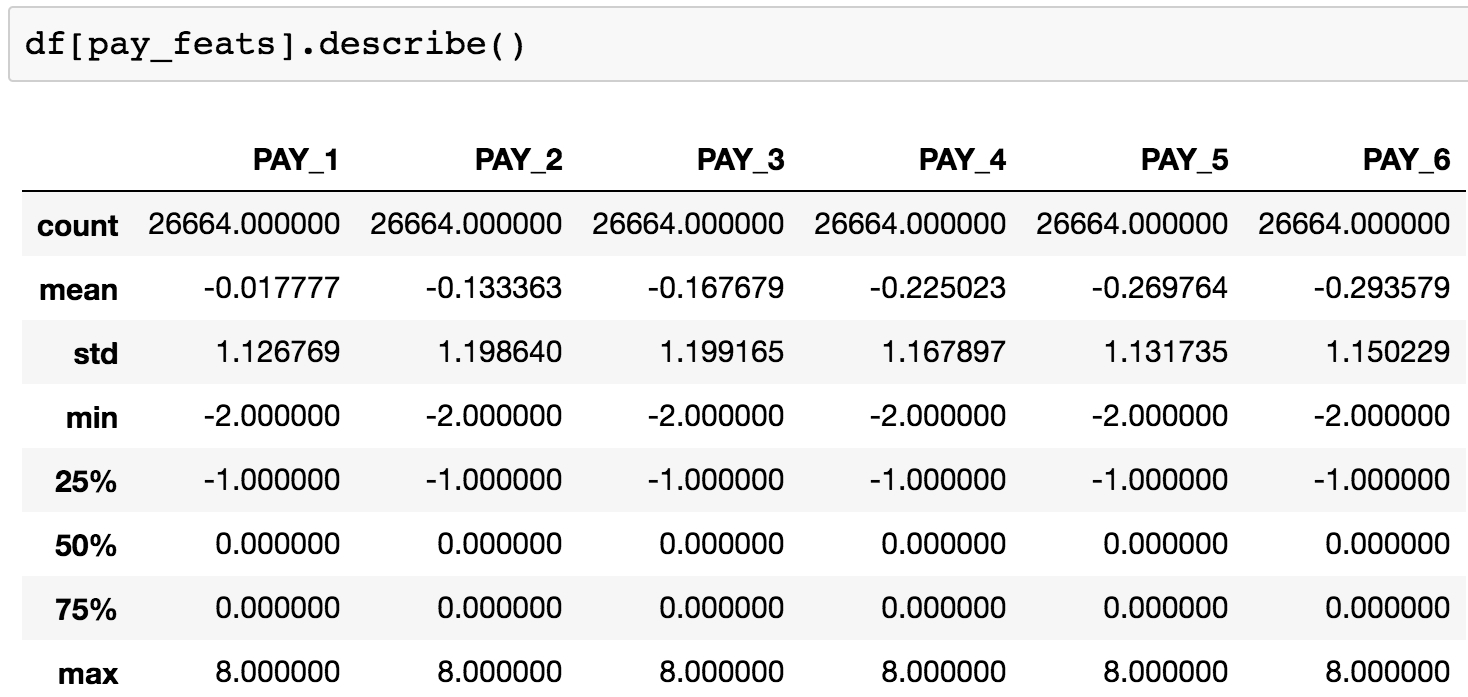Figure 1.55: Summary statistics of payment status features

Here, we observe that the range of values is the same for all of these features: -2, -1, 0, ... 8. It appears that the value of 9, described in the data dictionary as "payment delay for nine months and above", is never observed.

We have already clarified the meaning of all of these levels, some of which were not in the original data dictionary. Now let's look again at the value_counts() of PAY_1, now sorted by the values we are counting, which are the index of this Series:

df[pay_feats].value_counts().sort_index()

This should produce the following output: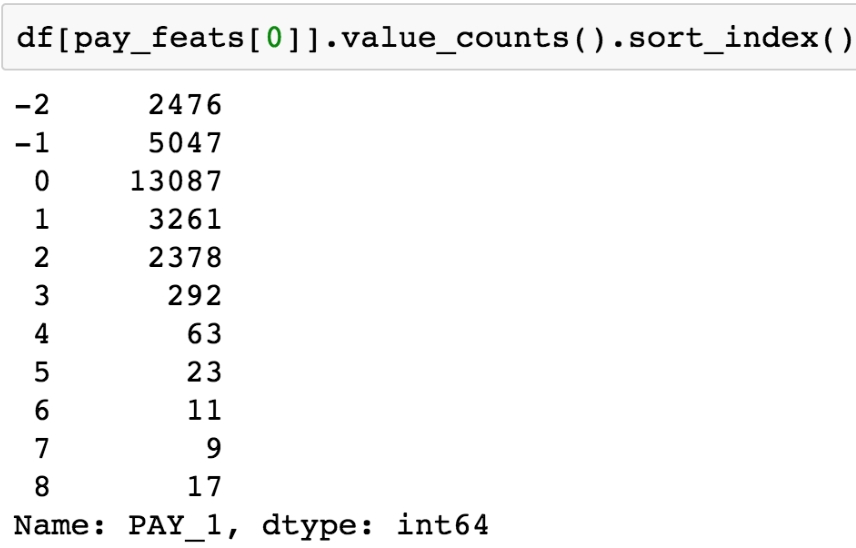Figure 1.56: Value counts of the payment status for the previous month

Compared to the positive integer values, most of the values are either -2, -1, or 0, which correspond to an account that was in good standing last month: not used, paid in full, or made at least the minimum payment.

Notice that, because of the definition of the other values of this variable (1 = payment delay for one month; 2 = payment delay for two months, and so on), this feature is sort of a hybrid of categorical and numerical features. Why should no credit usage correspond to a value of -2, while a value of 2 means a 2-month late payment, and so forth? We should acknowledge that the numerical coding of payment statuses -2, -1, and 0 constitute a decision made by the creator of the dataset on how to encode certain categorical features, which were then lumped in with a feature that is truly numerical: the number of months of payment delay (values of 1 and larger). Later on, we will consider the potential effects of this way of doing things on the predictive capability of this feature.

For now, we will simply continue to explore the data. This dataset is small enough, with 18 of these financial features and a handful of others, that we can afford to individually examine every feature. If the dataset had thousands of features, we would likely forgo this and instead explore dimensionality reduction techniques, which are ways to condense the information in a large number of features down to a smaller number of derived features, or, alternatively, methods of feature selection, which can be used to isolate the important features from a candidate field of many. We will demonstrate and explain some feature selection techniques later. But on this dataset, it's feasible to visualize every feature. As we know from the last chapter, a histogram is a good way to get a quick visual interpretation of the same kind of information we would get from tables of value counts. You can try this on the most recent month's payment status features with df[pay_feats].hist(), to produce this: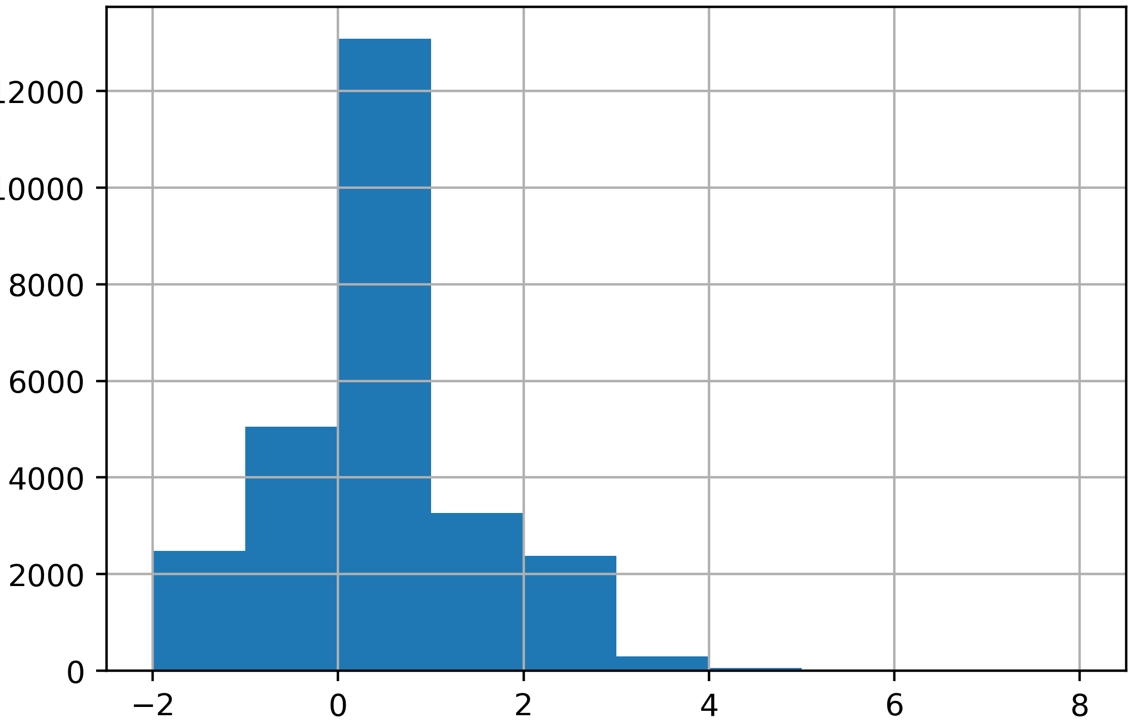Figure 1.57: Histogram of PAY_1 using default arguments

Now we're going to take an in-depth look at how this graphic is produced and consider whether it is as informative as it should be. A key point about the graphical functionality of pandas is that pandas plotting actually calls matplotlib under the hood. Notice that the last available argument to the pandas .hist() method is **kwds, which the documentation indicates are matplotlib keyword arguments.

Note

Looking at the matplotlib documentation for matplotlib.pyplot.hist shows additional arguments you can use with the pandas .hist() method, such as the type of histogram to plot (see https://matplotlib.org/api/_as_gen/matplotlib.pyplot.hist.html for more details). In general, to get more details about plotting functionality, it's important to be aware of matplotlib, and in some scenarios, you will want to use matplotlib directly, instead of pandas, to have more control over the appearance of plots.

You should be aware that aware that pandas uses matplotlib, which in turn uses NumPy. When plotting histograms with matplotlib, the numerical calculation for the values that make up the histogram is actually carried out by the NumPy .histogram function. This is a key example of code reuse, or "not reinventing the wheel". If a standard functionality, such as plotting a histogram, already has a good implementation in Python, there is no reason to create it anew. And the if mathematical operation to create the histogram data for the plot is already implemented, this should be leveraged as well. This shows the interconnectedness of the Python ecosystem.

We'll now address a couple of key issues that arise when calculating and plotting histograms.

Number of bins

Histograms work by grouping together values into what are called bins. The number of bins is the number of vertical bars that make up the discrete histogram plot we see. If there are a large number of unique values on a continuous scale, such as the histogram of ages we viewed earlier, histogram plotting works relatively well "out of the box", with default arguments. However, when the number of unique values is close to the number of bins, the results may be a little misleading. The default number of bins is 10, while in the PAY_1 feature, there are 11 unique values. In cases like this, it's better to manually set the number of histogram bins to the number of unique values.

In our current example, since there are very few values in the higher bins of PAY_1, the plot may not look much different. But in general, this is important to keep in mind when plotting histograms.

Bin edges

The locations of the edges of the bins determine how the values get grouped in the histogram. Instead of indicating the number of bins to the plotting function, you could alternatively supply a list or array of numbers for the bins keyword argument. This input would be interpreted as the bin edge locations on the x axis. The way values are grouped into bins in matplotlib, using the edge locations, is important to understand. All bins, except the last one, group together values as low as the left edge, and up to but not including values as high as the right edge. In other words, the left edge is closed but the right edge is open for these bins. However, the last bin includes both edges; it has a closed left and right edge. This is of more practical importance when you are binning a relatively small number of unique values that may land on the bin edges.

For control over plot appearance, it's usually better to specify the bin edge locations. We'll create an array of 12 numbers, which will result in 11 bins, each one centered around one of the unique values of PAY_1:

pay_1_bins = np.array(range(-2,10)) - 0.5
pay_1_bins

The output shows the bin edge locations: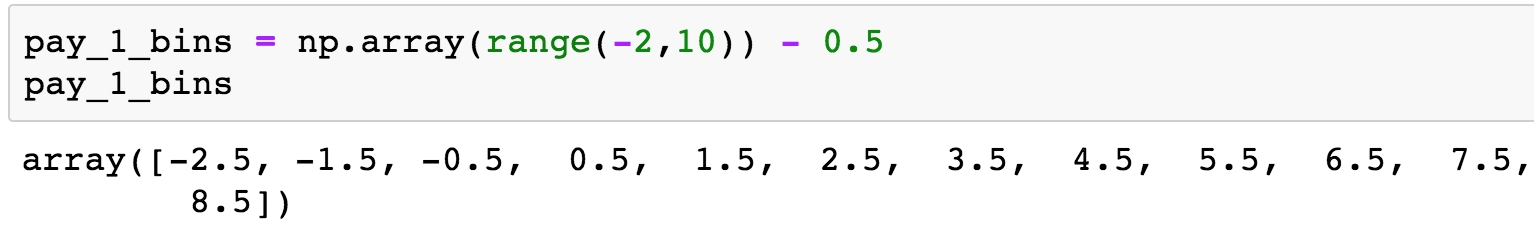Figure 1.58: Specifying histogram bin edges

As a final point of style, it is important to always label your plots so that they are interpretable. We haven't yet done this manually, because in some cases, pandas does it automatically, and in other cases, we simply left the plots unlabeled. From now on, we will follow best practice and label all plots. We use the xlabel and ylabel functions in matplotlib to add axis labels to this plot. The code is as follows:

df[pay_feats].hist(bins=pay_1_bins)
plt.xlabel('PAY_1')
plt.ylabel('Number of accounts')

The output should look like this: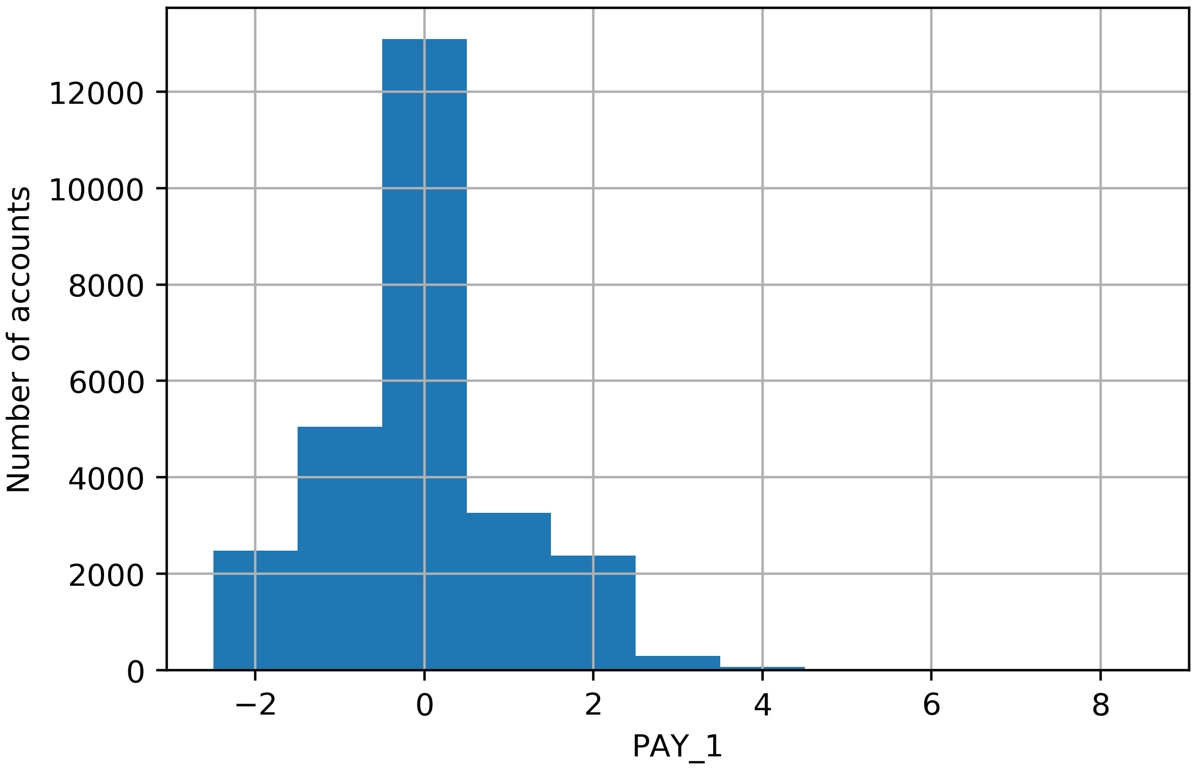Figure 1.59: A better histogram of PAY_1

While it's tempting, and often sufficient, to just call plotting functions with the default arguments, one of your jobs as a data scientist is to create accurate and representative data visualizations. To do that, sometimes you need to dig into the details of plotting code, as we've done here.

What have we learned from this data visualization?

Since we already looked at the value counts, this confirms for us that most accounts are in good standing (values -2, -1, and 0). For those that aren't, it's more common for the "months late" to be a smaller number. This makes sense; likely, most people are paying off their balances before too long. Otherwise, their account may be closed or sold to a collection agency. Examining the distribution of your features and making sure it seems reasonable is a good thing to confirm with your client, as the quality of these data underlie the predictive modeling you seek to do.

Now that we've established some good plotting style for histograms, let's use pandas to plot multiple histograms together, and visualize the payment status features for each of the last six months. We can pass our list of column names pay_feats to access multiple columns to plot with the .hist() method, specifying the bin edges we've already determined, and indicating we'd like a 2 by 3 grid of plots. First, we set the font size small enough to fit between these subplots. Here is the code for this:

mpl.rcParams['font.size'] = 4
df[pay_feats].hist(bins=pay_1_bins, layout=(2,3))

The plot titles have been created automatically for us based on the column names. The y axes are understood to be counts. The resulting visualizations are as follows: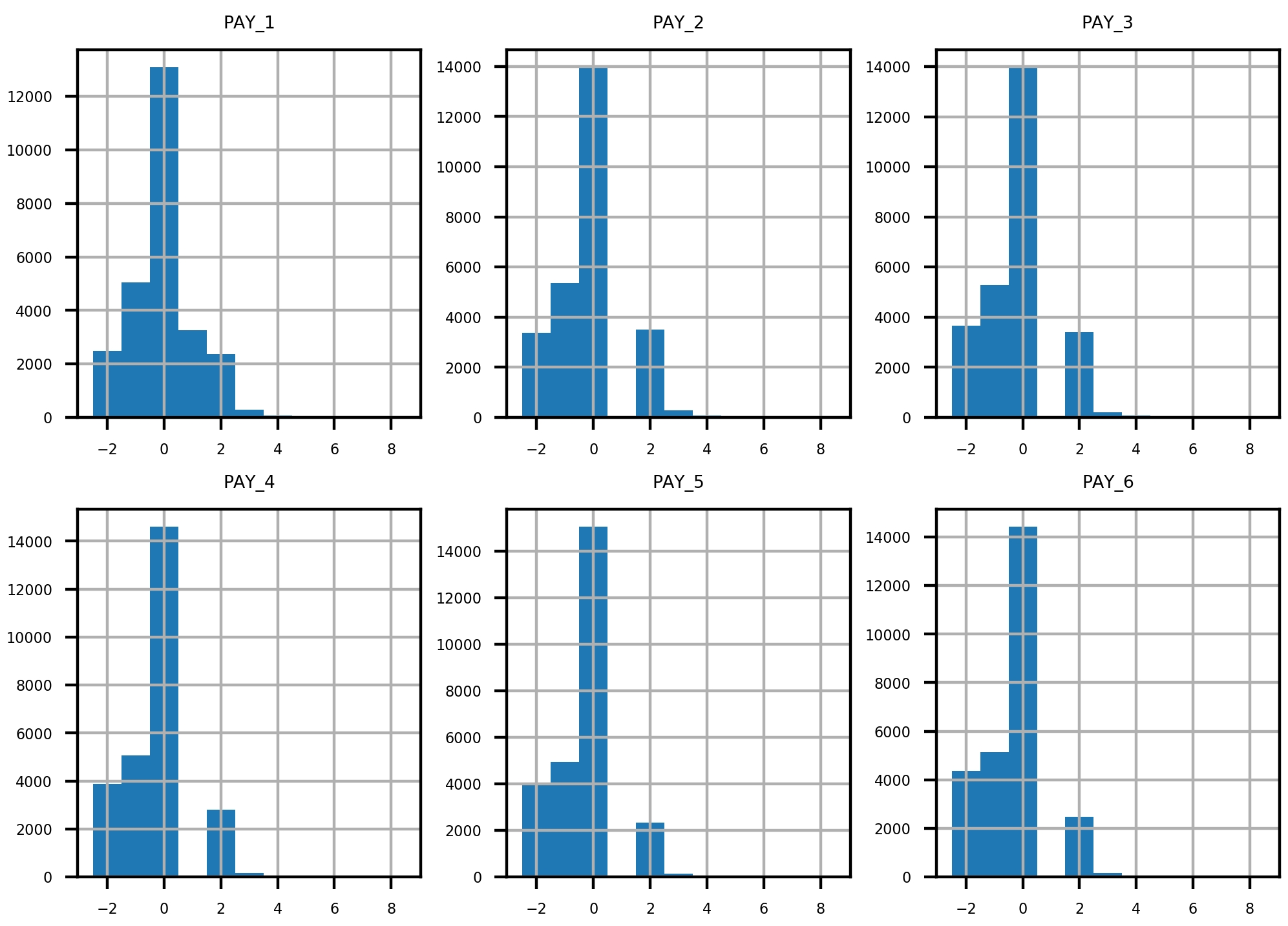Figure 1.60: Grid of histogram subplots

We've already seen the first of these, and it makes sense. What about the rest of them? Remember the definitions of the positive integer values of these features, and what each feature means. For example, PAY_2 is the repayment status in August, PAY_3 is the repayment status in July, and the others go further back in time. A value of 1 means payment delay for 1 month, while a value of 2 means payment delay for 2 months, and so forth.

Did you notice that something doesn't seem right? Consider the values between July (PAY_3) and August (PAY_2). In July, there are very few accounts that had a 1-month payment delay; this bar is not really visible in the histogram. However, in August, there are suddenly thousands of accounts with a 2-month payment delay. This does not make sense: the number of accounts with a 2-month delay in a given month should be less than or equal to the number of accounts with a 1-month delay in the previous month. Let's take a closer look at accounts with a 2-month delay in August and see what the payment status was in July. We can do this with the following code, using a Boolean mask and .loc, as shown in the following snippet:

The output of this should appear as follows: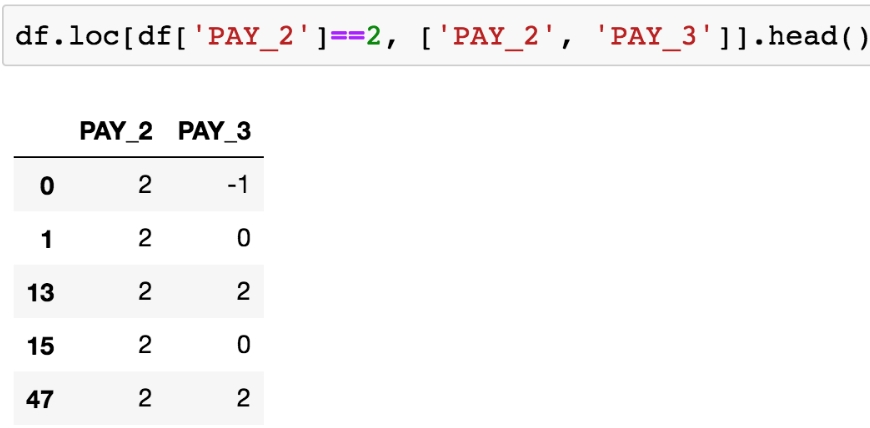Figure 1.61: Payment status in July (PAY_3) of accounts with a 2-month payment delay in August (PAY_2)

From Figure 1.61, it's clear that accounts with a 2-month delay in August have nonsensical values for the July payment status. The only way to progress to a 2-month delay should be from a 1-month delay the previous month, yet none of these accounts indicate that.

When you see something like this in the data, you need to either check the logic in the query used to create the dataset or contact the person who gave you the dataset. After double-checking these results, for example using .value_counts() to view the numbers directly, we contact our client to inquire about this issue.

The client lets us know that they had been having problems with pulling the most recent month of data, leading to faulty reporting for accounts that had a 1-month delay in payment. In September, they had mostly fixed these problems (although not entirely; that is why there were missing values in the PAY_1 feature, as we found). So, in our dataset, the value of 1 is underreported in all months except for September (the PAY_1 feature). In theory, the client could create a query to look back into their database and determine the correct values for PAY_2, PAY_3, and so on up to PAY_6. However, for practical reasons, they won't be able to complete this retrospective analysis in time for us to receive it and include it in our analysis.

Because of this, only the most recent month of our payment status data is correct. This means that, of all the payment status features, only PAY_1 is representative of future data, those that will be used to make predictions with the model we develop. This is a key point: a predictive model relies on getting the same kind of data to make predictions that it was trained on. This means we can use PAY_1 as a feature in our model, but not PAY_2 or the other payment status features from previous months.

This episode shows the importance of a thorough examination of data quality. Only by carefully combing through the data did we discover this issue. It would have been nice if the client had told us up front that they were having reporting issues over the last few months, when our dataset was collected, and that the reporting procedure was not consistent during that time period. However, ultimately it is our responsibility to build a credible model, so we need to be sure we believe the data is correct, by making this kind of careful examination. We explain to the client that we can't use the older features since they are not representative of the future data the model will be scored on (that is, make predictions on future months), and politely ask them to let us know of any further data issues they are aware of. There are none at this time.

Activity 1: Exploring Remaining Financial Features in the Dataset

In this activity, you will examine the remaining financial features in a similar way to how we examined PAY_1, PAY_2, PAY_3, and so on. In order to better visualize some of these data, we'll use a mathematical function that should be familiar: the logarithm. You'll use pandas to apply, which serves to apply any function to an entire column or DataFrame in the process. Once you complete the activity, you should have the following set of histograms of logarithmic transformations of non-zero payments: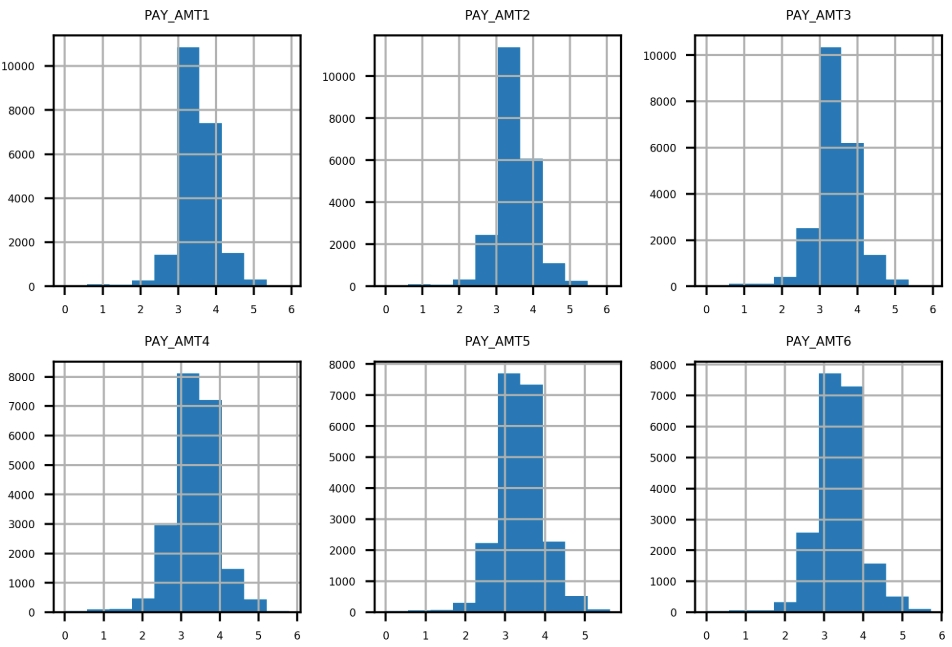Figure 1.62: Expected set of histograms

Perform the following steps to complete the activity:

Note

The code and the resulting output graphics for this exercise have been loaded into a Jupyter Notebook that can be found here: http://bit.ly/2TXZmrA.

1. Create lists of feature names for the remaining financial features.

2. Use .describe() to examine statistical summaries of the bill amount features. Reflect on what you see. Does it make sense?

3. Visualize the bill amount features using a 2 by 3 grid of histogram plots.

Hint: You can use 20 bins for this visualization.

4. Obtain the .describe() summary of the payment amount features. Does it make sense?

5. Plot a histogram of the bill payment features similar to the bill amount features, but also apply some rotation to the x-axis labels with the xrot keyword argument so that they don't overlap. In any plotting function, you can include the xrot=<angle> keyword argument to rotate x-axis labels by a given angle in degrees. Consider the results.

6. Use a Boolean mask to see how many of the payment amount data are exactly equal to 0. Does this make sense given the histogram in the previous step?

7. Ignoring the payments of 0 using the mask you created in the previous step, use pandas .apply() and NumPy's np.log10() to plot histograms of logarithmic transformations of the non-zero payments. Consider the results.

Hint: You can use .apply() to apply any function, including log10, to all the elements of a DataFrame or a column using the following syntax: .apply(<function_name>).

Note

The solution to this activity can be found on page 324.

Summary

This was the first chapter in our book, Data Science Projects with Python. Here, we made extensive use of pandas to load and explore the case study data. We learned how to check for basic consistency and correctness by using a combination of statistical summaries and visualizations. We answered such questions as "Are the unique account IDs truly unique?", "Is there any missing data that has been given a fill value?", and "Do the values of the features make sense given their definition?"

You may notice that we spent nearly all of this chapter identifying and correcting issues with our dataset. This is often the most time consuming stage of a data science project. While it is not always the most exciting part of the job, it gives you the raw materials necessary to build exciting models and insights. These will be the subjects of most of the rest of this book.

Mastery of software tools and mathematical concepts is what allows you execute data science projects, at a technical level. However, managing your relationships with clients, who are relying on your services to generate insights from their data, is just as important to a successful project. You must make as much use as you can of your business partner's understanding of the data. They are likely going to be more familiar with it than you, unless you are already a subject matter expert on the data for the project you are completing. However, even in that case, your first step should be a thorough and critical review of the data you are using.

In our data exploration, we discovered an issue that could have undermined our project: the data we had received was not internally consistent. Most of the months of the payment status features were plagued by a data reporting issue, included nonsensical values, and were not representative of the most recent month of data, or the data that would be available to the model going forward. We only uncovered this issue by taking a careful look at all of the features. While this is not always possible in different projects, especially when there is a very large number of features, you should always take the time to spot check as many features as you can. If you can't examine every feature, it's useful to check a few of every category of feature (if the features fall into categories, such as financial or demographic).

When discussing data issues like this with your client, make sure you are respectful and professional. The client may simply have forgotten about the issue when presenting you with the data. Or, they may have known about it but assumed it wouldn't affect your analysis for some reason. In any case, you are doing them an essential service by bringing it to their attention and explaining why it would be a problem to use flawed data to build a model. You should back up your claims with results if possible, showing that using the incorrect data either leads to decreased, or unchanged, model performance. Or, alternatively, you could explain that if only a different kind of data would be available in the future, compared to what's available now for training a model, the model built now will not be useful. Be as specific as you can, presenting the kinds of graphs and tables that we used to discover the data issue here.

In the next chapter, we will examine the response variable for our case study problem, which completes the initial data exploration. Then we will start to get some hands-on experience with machine learning models and learn how we can decide whether a model is useful or not.